Login

### Courses

 Syllabus: Pre-requisite: None Single Variable Calculus: Real number system: Completeness axiom, density of rationals (irrationals) in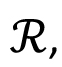, convergence of a sequence, Sandwich theorem, Monotone sequences, Cauchy Criterion, Subsequence, Every bounded sequence has a convergent subsequence, convergence of a sequence satisfying Cauchy criterion, Limits and Continuity of functions, Boundedness of a continuous function on [a, b], Existence of max of a continuous function on [a, b], Intermediate value property, Differentiability, Necessary condition for local maxima, Rolles theorem and Mean Value theorem, Cauchy mean value theorem, L `Hospital rule, Fixed point iteration method (Picard's method), Newton's method, Increasing and decreasing function, Convexity, Second derivative test for max and min, Point of Inflection, Curve Sketching, Taylor's theorem and remainder, Convergence of series, Geometric and Harmonic Series, Absolute convergence, Comparison test, Cauchy Condensation test: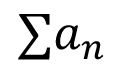converges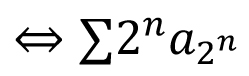converges for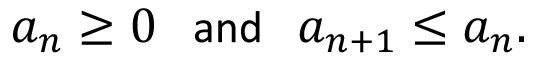Example. Ratio test, Root test, Examples, Leibniz' theorem, Power series, Radius of convergence, Taylor Series, Maclaurin Series, Introduction to Riemann Integration, Integrability, The Integral existence theorem for continuous functions and monotone functions, Elementary properties of integral, Fundamental theorems of Calculus, Trapezoidal approximation, Simpson's Rule, Improper integral of first and second kind, Comparison test, Absolute convergence, Application of definite integral, Area between two curves, Polar coordinates, Graphs of polar coordinates, Area between two curves when their equations are given in polar coordinates, Volumes by slicing, Volumes by Shells and Washers, Length of a curve, Area of surface of revolution, Pappus's theorem, Review of vector algebra, Equations of lines and planes, Continuity and Differentiability of vector functions, Arc length for space curves, Unit tangent vector, Unit normal and curvature to plane and space curves, Binormal, Functions of several variables, Continuity, Partial derivatives, Differentiability, Differentiability implies continuity, Increment theorem, Chain rule, Gradient, Directional derivatives, Tangent plane and Normal line, Mixed derivative theorem, Mean value theorem, Minima and Saddle point, Necessary and sufficient conditions for Maxima, minima and Saddle point, The method of Lagrange multipliers, Double Integral, Fubini's theorem, Volumes and Areas, Change of variable in double integral. Special cases: Polar coordinates, Triple integral, Applications, Change of variable in triple integral. Special cases: Cylindrical and Spherical coordinates, Surface area, Surface integral, Line integrals, Green's theorem, Vector fields Divergence and Curl of a vector field, Stoke's theorem, The divergence theorem. Reference materials: Thomas and Finney: "Calculus and Analytical Geometry", 9th Edition, Addison and Wesley Publishing Company. Credits: 11

 Syllabus: Pre-requisite: MTH 101 Linear Algebra: Matrices, System of linear equations, Gauss elimination method, Elementary matrices, Invertible matrices, Gauss-Jordon method for finding inverse of a matrix, Determinants, Basic properties of determinants. Cofactor expansion, Determinant method for finding inverse of a matrix, Cramer's Rule, Vector space, Subspace, Examples, Linear span, Linear independence and dependence, Basis, Dimension, Extension of a basis of a subspace, Intersection and sum of two subspace, Examples. Linear transformation, Kernel and Range of a linear map, Rank-Nullity Theorem. Rank of a matrix, Row and column spaces, Solvability of system of linear equations, some applications Inner product onCauchy-Schwartz inequality, Orthogonal basis, Gram-Schmidt orthogonalization process. Orthogonal projection, Orthogonal complement, Projection theorem, Fundamental subspaces and their relations, Applications (Least square solutions and least square fittings). Eigen-values, Eigen-Vectors, Characterization of a diagonalizable matrix. Diagonalization: Example, An application. Diagonalization of a real symmetric matrix. Representation of real linear maps by matrices (optional) Ordinary differential equations: Introduction to DE, Order of DE, First Order ODE F(x,y,y')=0. Concept of solution (general solution, singular solution, implicit solution etc.), Geometrical interpretations (direction fields, isoclines), Separable form, Reduction to separable form, Exact equations, Integrating factors (of the form F(x) and F(y)). Linear equations, Bernoulli equation, orthogonal trajectories. Picard's existence and uniqueness theorem (without proof), Picard's iteration method. Numerical methods: Euler's method, improved Euler's method. Second order linear ODE: fundamental system and general solutions of homogeneous equations, Wronskian, reduction of order. Characteristic equations: real distinct roots, complex roots, repeated roots. Non-homogeneous equations: undetermined coefficients. Non-homogeneous equations: variation of parameters. Extension to higher order differential equations, Euler-Cauchy equation. Power series solutions: ordinary points (Legendre equation). Power series solutions: regular singular points (Bessel equation), Frobenius method, indicial equations. Legendre polynomials and properties, Bessel functions and properties, Sturm comparison theorem, Sturm-Liouville boundary value problems, orthogonal functions. Laplace transform: Laplace and inverse Laplace transforms, first shifting theorem, existence, transforms of derivative and integral. Laplace transform: Differentiation and integration of transforms, unit step function, Second shifting theorem. Laplace transform: Convolution and applications, initial value problems. Reference materials: G. Strang: Linear Algebra, Introduction to linear algebra, 41 Edition, Wellesley Cambridge Press. G. F. Simmons: Ordinary Differential Equations, Differential equations with applications and historical notes, 2nd Edition. Credits: 11

 Syllabus: Pre-requisite: MTH 101, None for M.Sc. 2 yr Probability:- Axiomatic definition, properties, conditional probability, Bayes' rule and independence of events. Random variables, distribution function, probability mass and density functions, expectation, moments, moment generating function, Chebyshev's inequality. Special distributions; Bernoulli, binomial, geometric, negative binomial, hypergeometric, Poisson, exponential, gamma, Weibull, beta, Cauchy, double exponential, normal. Reliability and hazard rate, reliability of series and parallel systems. Joint distributions, marginal and conditional distributions, moments, independence of random variables, covariance and correlation. Functions of random variables. Weak Law of large numbers and Central limit theorems. Statistics:- Descriptive statistics, graphical representation of the data, measures of location and variability. Population, sample, parameters. Point estimation; method of moments, maximum likelihood estimator, unbiasedness, consistency. Confidence intervals for mean, difference of means, proportions. Testing of hypothesis; Null and Alternate hypothesis, Neyman Pearson fundamental lemma, Tests for one sample and two sample problems for normal populations, tests for proportions. Reference materials: Robert V. Hogg, J.W. McKean, and Allen T. Craig: Introduction to Mathematical Statistics, Seventh Edition, Pearson Education, Asia. Edward J Dudewicz and Satya N. Mishra: Modern Mathematical Statistics, Wiley. Credits: 11

 Syllabus: Pre-requisite: MTH 101 Complex Numbers, Polar form, De-Moivre's formula, convergent sequence, continuity, Complex differentiation, Cauchy-Riemann equation, Applications, Analytic functions and Power series, Derivative of a power series, Exponential function, Logarithmic function and trigonometric functions, Contour and Contour integral, Anti-derivative, ML inequality, Cauchy's theorem, Cauchy Integral formula, examples, Evolution of contour integrals, Derivatives of analytic functions, Cauchy's estimate, Liouville theorem, Fundamental theorem of Algebra, Morera's theorem (without proof), Taylor's theorem, Examples, Computation of Taylor's series. Zeros of Analytic functions. Identity theorem, Uniqueness theorem, Applications, Maximum modulus principle, Laurent series, Computation of Laurent expansion, Cauchy residue theorem, Poles, Residue at a pole, Examples, Evaluation of real improper integrals of different forms, Linear fractional transformations. Reference materials: E. Kreyszig, Advanced Engineering Mathematics. R. V. Churchill and J. W. Brown, Complex Variables and Applications. Credits: 6

 Syllabus: Pre-requisite: MTH 102 Introduction to PDE, Linear, non-linear (semi-linear quasilinear) examples, Order of a PDE, Strum-Liouville boundary values problems: Introduction, examples, orthogonal functions, Strum-Liouville expansion, Fourier series and its convergence, Fourier series of arbitrary period, Sine and Cosine series. Half-range expansion, Fourier integrals, Fourier-Legendre series, Fourier-Bessel series, First order (linear and semi-linear) PDEs, interpretation, method of characteristics, general solutions, First order quasi-linear PDEs, interpretation, method of characteristics, general solutions, Classification of 2nd order PDEs, Canonical form, hyperbolic equations, parabolic equations and elliptic equations, Wave equations, D'Alemberts formula, Duhamel's principle, Solutions for initial boundary value problem. Heat equations, Uniqueness and maximum principle, applications, Solutions for initial boundary value problem. Laplace and Poisson equations, uniqueness and maximum principle for Dirichlet problem, Boundary value problems in 2D (rectangular, polar), Boundary value problems in 3D (rectangular, cylindrical, spherical). Reference materials: E. Kreyszig, Advanced Engineering Mathematics. Credits: 6

Departmental Courses

 Syllabus: Pre-requisite: MTH102, None for M.Sc. 2yr Matrices: Elementary matrices, invertible matrices, Gauss-Jordon method, determinant, Systems of linear equations and Cramer's Rule. Vector spaces: Fields, Vector spaces over a field, subspaces, Linear independence and dependence, existence of basis, coordinates, dimension. Linear Transformations: Rank Nullity Theorem, isomorphism, matrix representation of linear transformation, change of basis, similar matrices, linear functional and dual space. Inner product spaces: Cauchy-Schwarz's inequality, Gram-Schmidt orthonormalization, orthonormal basis, orthogonal projection, projection theorem, four fundamental subspaces and their relations (relation between null space and row space; relation between null space of the transpose and the column space). Diagonalization: Eigenvalues and eigenvectors, diagonalizability, Invariant subspaces , adjoint of an operator, normal, unitary and self adjoint operators, Schur's Lemma, diagonalization of normal matrices, spectral decompositions and spectral theorem, applications of spectral theorem, Cayley-Hamilton theorem, primary decomposition theorem, Jordon canonical form, minimal polynomials, Introduction to bilinear and Quadratic forms: Bilinear and quadratic forms, Sylvester's law of inertia. Some applications: Lagrange interpolation, LU,QR and SVD decompositions, least square solutions, least square fittings, pseudo inverses. Reference materials: Kenneth Hoffman and Ray Kunze: Linear Algebra, PHI publication. Gilbert Strang: Linear Algebra and Its Applications, 4th edition. Sheldon Axler: Linear Algebra Done Right, UTM, Springer. Credits: 11

 Syllabus: Pre-requisite: MTH 102, None for M.Sc. 2yr Basic set theory: Unions, Intersections, Pairs, Powers, Relations and Functions, Partial Orders, Numbers, Peano's Axioms, Mathematical Induction, Finite and Infinite Sets, Families of sets: Product of sets(finite and infinite), More on relations and functions, Schroder-Bernstein Theorem, Countable and Uncountable Sets, Axiom of Choice, Zorn's Lemma, Cardinals and ordinals, Integers, Divisibility in Integers, GCD, Bezout's identity, modular arithmetic, Chinese remainder theorem, Fermat's little theorem, Euler Phi-function, Permutation, Combinations, Circular permutations, Binomial and Multinomial theorems, Solutions in nonnegative integers, Balls into Boxes-Pigeon-hole Principle, Inclusion-Exclusion Principle, Recurrence Relations, Generating Functions, generating functions from recurrence relation. Reference materials: Kenneth Rosen: Discrete Mathematics and Its Applications, McGraw Hill Education; 7th edition. Donald Knuth, Oren Patashnik, and Ronald Graham: Concrete Mathematics, Addison-Wesley Professional. David M. Burton: Elementary Number Theory. Credits: 11

 Syllabus: Pre-requisite: MTH102, None for M.Sc. 2yr Group theory: Binary operation, and its properties, Definition of a group, Groups as symmetries, Examples: cyclic, dihedral, symmetric, matrix groups, Subgroups, Cosets, normal subgroups and quotient groups, Conjugacy classes, Lagrange's theorem, The isomorphism theorems, Direct and semi-direct products, Group automorphisms, Symmetric group and alternating group, Actions of groups on sets, Cayley's theorem, orbit and stabilizers, Class equations, p-groups, Sylow's theorem and applications: simplicity of groups, Classification of finite abelian groups. (Time permitting: Finitely-generated abelian groups, Free groups, Composition series, Jordan-Hölder theorem, Nilpotent and solvable groups). Ring Theory: Definition and examples, Ring homomorphism, Ideals and Quotient rings, Chinese Remainder Theorem, Integral Domain and quotient fields, Unique factorization domain, Principal Ideal domain, Euclidean domain, Gauss lemma, Polynomial Rings, Irreducibility of Polynomials, Ring of Gaussian Integers. Reference materials: J. Gallian: Contemporary Abstract Algebra, Narosa books Pvt. Ltd. I. N. Herstein: Topics in Algebra, Wiley. D. S. Dummit and R.M. Foote, Abstract Algebra, Wiley. M. Artin, Algebra, PHI. N. Jacobson, Basic Algebra I, Basic Algebra II, Dover Publications. Credits: 11

 Syllabus: Pre-requisite: MTH101, MTH 102, None for M.Sc. 2yr Fourier series, Convolutions, Good kernels, Cesaro and Abel Summable, Convergence of Fourier series: Mean square, Pointwise convergence, Applications: Weyl equi-distribution, Isoperimetric inequality, Construction of continuous but nowhere differentiable function, Finite Fourier Analysis: on abelian groups, Characters as a total family, Fourier inversion and Plancherel formula, Dirichlet's Theorem: A little elementary number theory, Dirichlet's theorem, Proof of the theorem. Reference materials: E. M. Stein and R. Shakarchi: Fourier analysis: An Introduction. Credits: 11

 Syllabus: Pre-requisite: MTH 102, None for M.Sc. 2 yr Divisibility, Primes, fundamental theorem of arithmetic, Euclidean algorithm, Congruence and modular arithmetic, Chinese remainder theorem, Roots of unity, Quadratic reciprocity, Binary quadratic forms, Some Diophantine equations, Some arithmetic functions, Distribution of prime numbers, Bertrand's postulate, the partition function, Dirichlet Series, Riemann Zeta function. Reference materials: I. Niven, H. S. Zuckerman and H. L. Montgomery. An Introduction to the Theory of Numbers, Wiley, 1991 David M. Burton, Elementary Number Theory. McGraw Hill Education, 2012. K. Ireland, and M. Rosen. A Classical Introduction to Modern Number Theory. GTM-84, Springer, 1990. G. H. Hardy and E. M. Wright. An Introduction to the Theory of Numbers. Oxford University Press, 1960. Credits: 11

 Syllabus: Pre-requisite: MTH 101, None for M.Sc. 2 yr Real Number system: Completeness property. Countable and Uncountable. Metric Spaces: Metric spaces, Examples:Limit, Open sets, Convergence of a sequence, Closed sets, Continuity. Completeness: Complete metric space, Nested set theorem, Baire category theorem, Applications. Compactness: Totally bounded, Characterizations of compactness, Finite intersection property, Continuous functions on compact sets, Uniform continuity. Connectedness: Characterizations of connectedness, Continuous functions on connected sets, Path connected. Riemann integration: Definition and existence of integral, Fundamental theorem of calculus, Set of measure zero, Cantor set, Characterization of integrable functions. Convergence of sequence and series of functions: Pointwise and uniform convergence of functions, Series of functions, Power series, Dini's theorem, Ascoli's theorem, Continuous function which is nowhere differentiable, Weierstrass approximation theorem. Reference materials: N. L. Carothers, Real Analysis. R. R. Goldberg, Methods of Real Analysis. W. Rudin, Principles of Mathematical Analysis. Credits: 11

 Syllabus: Pre-requisite: MTH 102, MTH 202 for M.Sc. 2 yr Some basics of set theory: Relations, Partitions, Functions and sets of functions. Families of sets, Cartesian products of families. Principles of weak and strong mathematical induction and their equivalence. Schroder-Bernstein theorem. Countable and uncountable sets. Cantor's theorem. Classical propositional calculus (PC): Syntax. Valuations and truth tables, Truth functions, Logical equivalence relation. Semantic consequence and satisfiability. Compactness theorem with application. Adequacy of connectives. Normal forms. Applications to Circuit design. Axiomatic approach to PC: soundness, consistency, completeness. Other proof techniques: Sequent calculus, Computer assisted formal proofs: Tableaux. Decidability of PC, Boolean algebras: Order relations. Boolean algebras as partially ordered sets. Atoms, Homomorphism, sub-algebra. Filters. Stone's representation (sketch). Completeness of PC with respect to the class of all Boolean algebras. Classical first order logic (FOL) and first order theories, Syntax. Satisfaction, truth, validity in FOL. Axiomatic approach, soundness. Computer assisted formal proofs: Tableaux. Consistency of FOL and completeness (sketch). Equality. Examples of first order theories with equality. Peano's arithmetic. Zermelo-Fraenkel axioms of Set theory. Axiom of choice, Well-ordering theorem, Zorn's lemma and their equivalence; illustrations of their use. Well-ordering principle and its equivalence with principles of weak and strong induction. Elementary model theory: Compactness theorem, Löwenheim-Skolem theorems. Completeness of first order theories, Isomorphism of models, Categoricity-illustrations through theories such as those of finite Abelian groups, dense linear orders without end points and Peano's arithmetic. Statements of Gödel's incompleteness theorems and un-decidability of FOL. Reference materials: J. Bridge: Beginning Model Theory: The Completeness Theorem and Some Consequences. Oxford Logic Guides, 1977. I. Chiswell and W. Hodges: Mathematical Logic. Oxford, 2007. R. Cori and D. Lascar: Mathematical Logic, Oxford, 2001. J. Goubalt-Larrecq and J. Mackie: Proof Theory and Automated Deduction, Kluwer, 1997. P. R. Halmos: Naive Set Theory, Springer, 1974. J. Kelly: The Essence of Logic, Pearson, 2011. A. Margaris, First Order Mathematical Logic, Dover, 1990. Credits: 11

 Syllabus: Pre-requisite: MTH 301 Topological spaces; open sets, closed sets, basis, sub-basis, closure, interior and boundary. Subspace topology. Continuous maps, open maps, closed maps, Homeomorphisms. Product Topology. Hausdorff spaces, Countability and separation axioms. Compact spaces and its properties, Locally compact spaces, one point compactification, Tychonoff's Theorem, Statement and Applications of Urysohn Lemma, Tietz extension theorem and Urysohn metrization theorem. Connectedness, path connectedness, components, its properties. Quotient Topology, various type of examples, cone, suspension, surfaces as quotient spaces. Group actions, orbit spaces. (Time permitting: Homotopy, Fundamental group, deformation retract, contractible spaces, simply connected spaces, computation of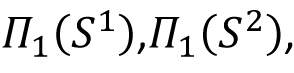,, Brouwer fixed point theorem.) Reference materials: J. R. Munkres: Topology: A First Course, Prentice-Hall, 1975. J. Dugundji: Topology, UBS, 1999. M. A. Armstrong: Basic Topology, Springer. G. F. Simmons: Introduction to Topology and Modern Analysis, Tata McGraw-Hill, 1963. Credits: 11

 Syllabus: Pre-requisite: MTH 301 Differentiation: Differentiable functions, Directional derivatives, Composition of differentiable functions and chain rule, Mean value inequalities. Inverse mapping theorem and Implicit mapping theorem. Regular value of differentiable maps, Lagrange multipliers. Higher order derivatives, Taylor's Theorem. Curves: Definition and examples of regular curves in R^2 and R^3. Definition of parametrised curves. Arc length of a regular curve and arc length parametrisation of regular curves. Curvature of plane curves and Frenet-Serret formula for regular space curves. Statement of Green's Theorem. Isoperimetric inequality for plane curves. Surfaces: Three equivalent definition of regular surfaces in R^3. Tangent planes. Differentiable functions on surfaces and differentiable maps between surfaces. Tangent Plane. Derivative of differentiable functions/maps on surfaces. First fundamental form. Local isometries. Gauss map, Weingartent map and second fundamental form. Principal curvatures, Gauss curvature and mean curvature of surfaces. Surfaces of revolution and classification of surfaces of revolution of constant curvature. Umbilic points on surface. Classification of totally umbilical surfaces. Hilbert's theorem on compact surfaces. Geodesics and examples. Reference materials: Tom M. Apostol: Mathematical Analysis, Narosa Publishing House, India. W. Rudin: Principles of Mathematical Analysis. Spivak: Calculus on manifolds, Springer. A Pressley: Elementary differential geometry, Springer India. M P do Carmo: Differential geometry of curves and surfaces, Prentice Hall. Credits: 11

 Syllabus: Pre-requisite(s): MTH 102 / None for M.Sc. 2 yr Approximations in Scientific computing, Error propagation and amplification, conditioning, stability and accuracy, computer arithmetic mathematical software and libraries, visualization, linear systems- existence and uniqueness, sensitivity and conditioning, Gaussian elimination, special linear systems, iterative methods, nonlinear equations, convergence rates, non-linear equations in one dimension, system of non-linear equations, eigenvalue problems, existence and uniqueness, sensitivity and conditioning, computing eigenvalues and eigenvectors, approximation and interpolation, Hermite and Spline interpolation, piecewise polynomial interpolation, numerical differentiation and integration, Chebyshev differentiation and FFT, Richardson extrapolation. Reference material(s): M Heath: Scientific Computing - An introductory Survey. Kendall E. Atkinson: An Introduction to Numerical Analysis. S. D. Conte & S. de Boor: Elementary Numerical Analysis: An Algorithmic Approach. J. Stoer and R. Bulirsch: Introduction to Numerical Analysis. Credits: 10

 Syllabus: Pre-requisite(s): MSO201, HSO 201/ Instructor's consent Limits of sequences of sets, σ-field of events. Probability measure, probability space. Random variables, induced probability space, probability distribution. Distribution function, decomposition theorem. Expectation and moments, inequalities. Various modes of convergence of sequences of random variables (in probability, almost surely, in rthmean). Convergence theorems for expectations of sequences of random variables (monotone convergence theorem, Fatou's lemma, dominated convergence theorem).Characteristic function and its properties, inversion formulae. Convergence of sequences of distribution functions, Helly-Bray theorems, convergence of moments. Independence of events and random variables, zero one laws. Convergence of series of independent random variables, Kolmogorov inequality, Kolmogorov three-series criterion, Khintchin's weak law of large numbers, Kolmogorov strong law of large numbers. Central limit theorems of Lindeberg-Levy, Liapounov and Lindeberg-Feller. Referencematerials: K.L.Chung: A Course in Probability Theory, Third Edition, Academic Press, 2001. B.R.Bhat: Modern Probability Theory, Third Edition, New Age International (P) Ltd, 2004. M. Loéve: Probability Theory-I, Graduate Text in Mathematics, Fourth Edition, Springer, 1977. Credits: 11

 Syllabus: Pre-requisite: None (Only for BS students) Credits: 4

 Syllabus: Pre-requisite: None (Only for BS students) Credits: 9

 Syllabus: Pre-requisite: None (Only for BS students) Credits: 9

 Syllabus: Pre-requisite: None (Only for BS students) Credits: 4

 Syllabus: Pre-requisite: None (Only for BS students) Credits: 5

 Syllabus: Pre-requisite: MTH302/Consent of Instructor Regular languages, Deterministic and non-deterministic finite automata, Closure properties, Languages that are and are not regular, State minimization in deterministic finite automata. Context-free languages, Closure properties, Parse-trees, Languages that are and are not Context-free, Pushdown automata. Turing machines, Turing computability, Church-Turing thesis, Halting problem, Some undecidable problems. Computational complexity, Classes P and NP, Completeness, Examples of NP complete problems. Reference materials: H. R. Lewis and C. H. Papadimitriou: Elements of the Theory of Computation, Prentice Hall, 1998. J. E. Hopcroft, R. Motwani, J. D. Ullman: Introduction to Automata Theory, Languages and Computation, Pearson Education, 2001. Credits: 9

 Syllabus: Pre-requisite: MTH301/Consent of Instructor Preliminaries to Complex analysis: Basic properties: convergence, compactness, connectedness; continuous functions, Holomorphic functions, power series, integration along curves and properties. Cauchy's theorem and its application: Goursat's theorem, local existence of primitives and Cauchy's theorem in a disc, evaluation of some integrals, Cauchy's integral formulas, Morera's theorem, sequence of holomorphic functions. Meromorphic functions and the Logarithm: Zeros and poles, the residue formula, singularities and meromorphic functions, the argument principle and applications, open mapping theorem, maximum modulus principle, Picard's little theorem, the complex logarithms, harmonic functions. Conformal mappings: Conformal equivalence: the disc and the upper half-plane; The Dirichlet problem in a strip, Schwartz lemma, automorphism of disc, automorphism of the upper half-plane, Montel Theorem, Riemann mapping theorem. Reference materials: L. V. Ahlfors: Complex analysis, McGraw-Hill international editions. John B. Conway: Functions of one complex variable, Springer International Student Edition. R. Narasimhan, Y. Nievergelt: Complex analysis in one variable, Birkhauser. Walter Rudin: Real and Complex analysis, McGraw-Hill international editions. E. M. Stein and R. Shakarchi: Complex Analysis, Princeton University Press. T. Gamelin: Complex analysis, Springer. Credits: 11

 Syllabus: Pre-requisite: MTH301/ Instructor's Consent Lebesgue measure on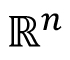: Introduction, outer measure, measurable sets, Lebesgue measure, regularity properties, non-measurable sets, measurable functions, Egorov's theorem, Lusin's theorem, Lebesgue Integration: Simple functions, Lebesgue integral of a bounded function over a set of finite measure, bounded convergence theorem, Integral of non-negative measurable functions, Fatou's lemma, monotone convergence theorem, general Lebesgue integral, Dominated convergence theorem, change of variable formula, Fubini's theorem.spaces: The Minkowski's inequality and Hölder's inequality, completeness of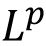and denseness results inDifferentiation and Integration: Functions of bounded variation, differentiation of an integral, Absolute continuity. Reference materials: G. de Barra: Measure theory and integration, Harwood Publishing Limited, Chichester, 2003. E. M. Stein and R. Shakarchi: Real analysis, measure theory, integration and Hilbert spaces, Princeton University Press. Walter Rudin: Real and Complex analysis, McGraw-Hill international editions. H. L. Royden and P. M. Fitzpatric, Real Analysis, 4th ed, Pearson, 2015. Credits: 11

 Syllabus: Pre-requisite: MTH301/Consent of Instructor Banach spaces, Riesz Lemma (On compactness of the unit ball in a normed linear space), Bounded linear maps on finite and infinite-dimensional normed linear spaces: Hahn Banach Theorem (geometric and extension forms), characterization of finite-dimensional normed linear spaces, Fundamental theorems on Banach spaces-Uniform Boundedness Principle, Closed Graph Theorem, Open Mapping Theorem. Dual spaces of some classical spaces, e.g.,Weak and Weak* convergence Banach Alaoglu Theorem. Hilbert spaces: Gram-Schmidt orthonormalization process, Bessel's inequality, orthonormal basis, Riesz Representation Theorem-Dual of a Hilbert space, Bounded operators on a Hilbert space: Adjoint of an operator, orthogonal projections, self-adjoint, normal and unitary operators Introduction to Banach Algebras-Spectrum of an operator, Spectral Theorem for compact self-adjoint operators, Spectral Theorem for self-adjoint operators (optional) Reference materials: J. B. Conway: A First Course in Functional Analysis Walter Rudin: Functional Analysis Rajendra Bhatia: Notes on Functional Analysis B. V. Limaye: Functional Analysis E. Kreyszig, Introductory Functional Analysis with Applications, Wiley, 2015. Credits: 11

 Syllabus: Pre-requisite: MSO 201, None for M.Sc. 2 yr Stats Definition and classification of general stochastic processes. Markov Chains: definition, transition probability matrices, classification of states, limiting properties. Markov Chains with Discrete State Space: Poisson process, birth and death processes. Renewal Process: renewal equation, mean renewal time, stopping time. Markov Process with Continuous State Space: Introduction to Brownian motion. Reference materials: Sheldon M Ross: Stochastic Processes, John Wiley and Sons, 1996. S Karlin and H M Taylor: A First Course in Stochastic Processes, Academic Press, 1975. Credits: 11

 Syllabus: Pre-requisite: MSO 201, None for M.Sc. 2 yr Stats Review of finite dimensional vector spaces (Null space and nullity), Linear dependence and independence, Matrix algebra, Rank of a Matrix, Inverse of a non-singular matrix. Hermite canonical forms, Generalized inverses, Moore-Penrose inverse, solution of linear equations, Projection and orthogonal projection matrices, Idempotent matrices. Real quadratic forms, reduction of pair of real symmetric matrices, Singular value decomposition. Extrema of a quadratic forms, Vector and matrix differentiation. Least squares theory and Gauss-Markoff theorem, Cochran's theorem and distribution of quadratic forms, test of single linear hypothesis and more than one hypothesis, ANOVA table, Confidence interval and regions, Power of F-test. Multiple comparisons and simultaneous confidence intervals. Reference materials: R. B. Bapat: Linear Algebra and Linear Models, Springer, London. David A. Herville: Matrix Algebra from a Statistician's Perspective, Springer-Verlag, New York. A. R. Rao and P. Bhimasankaram: Linear Algebra (Texts and Readings in Mathematics), Hindustan Book Agency. Gilbert Strang: Linear Algebra and its Applications, Cengage Learning. Debasis Sengupta and S. R. Jammalamadaka: Linear Models: An Integrated Approach, World Scientific. Credits: 11

 Syllabus: Pre-requisite: MSO 201, None for M.Sc. 2 yr Stats Simple and multiple linear regression, Polynomial regression and orthogonal polynomials, Test of significance and confidence intervals for parameters. Residuals and their analysis for test of departure from the assumptions such as fitness of model, normality, homogeneity of variances, detection of outliers, Influential observations, Power transformation of dependent and independent variables. Problem of multi-collinearity, ridge regression and principal component regression, subset selection of explanatory variables, Mallow's Cp statistic. Nonlinear regression, different methods for estimation (Least squares and Maximum likelihood), Asymptotic properties of estimators. Generalized Linear Models (GLIM), Analysis of binary and grouped data using logistic and log-linear models. Reference materials: Douglas C. Montgomery, Elizabeth A. Peck, G. Geoffrey Vining: Introduction to Linear Regression Analysis, Wiley, Low price Indian edition is available. Norman R. Draper, Harry Smith: Applied Regression Analysis, Low price Indian edition is available. C.R. Rao, H. Toutenburg, Shalabh, and C. Heumann: Linear Models and Generalizations - Least Squares and Alternatives, Springer, 2008. John F. Monahan: A Primer on Linear Models, CRC Press, 2008. Andre I. Khuri: Linear Model Methodology, CRC Press, 2010. H.D. Vinod and Aman Ullah: Recent advances in regression methods, Marcel Dekker. Credits: 11

 Syllabus: Pre-requisite: MSO 201, None for M.Sc. 2 yr Stats Principles of sample surveys; Simple, Stratified and unequal probability Sampling with and without replacement; ratio, product and regression method of estimation; systematic sampling; cluster and subsampling with equal unequal sizes; double sampling; sources of errors in surveys Reference materials: W.G. Cochran: Sampling Techniques, Wiley (Low price edition available) Parimal Mukhopadhyay: Theory and Methods of Survey Sampling, Prentice Hall of India P.V. Sukhatme, B.V Sukhatme, S. Sukhatme and C. Asok: Theory of Sample surveys with applications, IASRI, Delhi P.S.R.S. Rao: Sampling Methodologies and Applications, Chapman and Hall/ CRC M.N. Murthy: Sampling Theory and Methods, Statistical Publishing Society, Calcutta Z. Govindrajalu: Elements of sampling theory and methods, Prentice Hall Credits: 11

 Syllabus: Pre-requisite: MSO 201, None for M.Sc. 2 yr Stats Exponential families-introduction, canonical form, full rank; Sufficient statistic-sufficiency, Neyman Fisher factorization criterion, minimal sufficiency; Ancillary statistic; Completeness-completeness of family of distributions, completeness of statistic; Basu's theorem and its uses; Rao-Blackwell theorem and its implications; Unbiasedness-basic concepts, locally minimum variance unbiased estimator, uniformly minimum variance unbiased estimator, Lehmann- Scheffe's theorem and its importance; Methods for finding UMVUE-method of solving, Rao-Blackwellization; Non-parametric families and Hoeffding's U-statistic; Information inequality and lower bounds- Hammersley-Chapman-Robbins inequality, Fisher information, Cramer-Rao lower bond; Information inequality for multi-parameter case-information matrix, s-parameter exponential family, Bhattacharya system of lower bounds; Methods of estimation-MLE, MOME, MinMSE; Basic concepts in statistical hypotheses testing-simple and composite hypothesis, critical regions, Type-I and Type-II errors, size and power of a test; Neyman-Pearson lemma and its applications; Type of optimum tests and their construction using NP lemma- Most powerful test, uniformly most powerful test, unbiased test and uniformly most unbiased test; Monotone Likelihood ratio and testing with MLR property; Testing in one-parameter exponential families-one sided hypothesis, UMP and UMPU tests for different two-sided hypothesis; Testing in multi-parameter exponential families-tests with Neyman structure, UMP and UMPU similar size-tests; Likelihood Ratio test; Confidence intervals-pivotal functions, shortest expected length confidence interval, UMA and UMAU confidence intervals. Reference materials: John Rice: Mathematical Statistics and Data Analysis, 3rd edition Jun Shao: Mathematical Statistics, 2nd edition George Casella and Roger Berger: Statistical Inference, 2nd edition Credits: 11

 Syllabus: Pre-requisite: MSO 201, None for M.Sc. 2 yr Stats Exponential families-introduction, canonical form, full rank; Sufficient statistic-sufficiency, Neyman Fisher factorization criterion, minimal sufficiency; Ancillary statistic; Completeness-completeness of family of distributions, completeness of statistic; Basu's theorem and its uses; Rao-Blackwell theorem and its implications; Unbiasedness-basic concepts, locally minimum variance unbiased estimator, uniformly minimum variance unbiased estimator, Lehmann- Scheffe's theorem and its importance; Methods for finding UMVUE-method of solving, Rao-Blackwellization; Non-parametric families and Hoeffding's U-statistic; Information inequality and lower bounds- Hammersley-Chapman-Robbins inequality, Fisher information, Cramer-Rao lower bond; Information inequality for multi-parameter case-information matrix, s-parameter exponential family, Bhattacharya system of lower bounds; Methods of estimation-MLE, MOME, MinMSE; Basic concepts in statistical hypotheses testing-simple and composite hypothesis, critical regions, Type-I and Type-II errors, size and power of a test; Neyman-Pearson lemma and its applications; Type of optimum tests and their construction using NP lemma- Most powerful test, uniformly most powerful test, unbiased test and uniformly most unbiased test; Monotone Likelihood ratio and testing with MLR property; Testing in one-parameter exponential families-one sided hypothesis, UMP and UMPU tests for different two-sided hypothesis; Testing in multi-parameter exponential families-tests with Neyman structure, UMP and UMPU similar size-tests; Likelihood Ratio test; Confidence intervals-pivotal functions, shortest expected length confidence interval, UMA and UMAU confidence intervals. Reference materials: John Rice: Mathematical Statistics and Data Analysis, 3rd edition Jun Shao: Mathematical Statistics, 2nd edition George Casella and Roger Berger: Statistical Inference, 2nd edition Credits: 11

 Syllabus: Pre-requisite: MTH 102 / Instructor's consent Introduction to ODE; Existence and uniqueness of solution; Continuity and differentiability of solution w.r.t. initial condition and parameters; General theory of linear differential equations; Methods of solving non-homogeneous linear equations; Cauchy-Euler equation; Linear equations with periodic coefficients; System of linear differential equations; Stability theory for system of linear differential equations; Strum-Liouville boundary value problems, Oscillation theory; Green's function. Reference materials: Martin Brown, Differential Equations and Their Applications, Springer, 1992. S. L. Ross, Introduction to Ordinary Differential Equations, Wiley, 1980. Deo, Lakshmikantham, V Raghavendra, Textbook of Ordinary Differential Equations, Tata McGraw Hill, 1997. C. Y. Lin, Theory and Examples of Ordinary Differential Equations, World Scientific, 2011. Credits: 11

 Syllabus: Pre-requisite: MTH 421 / Instructor's consent Introduction to PDEs, First order quasilinear and nonlinear equations; Higher order equations and classifications; Solution of wave equations, Duhamel's principle and applications; Existence and uniqueness of solutions; BVPs for Laplace's and Poisson's equations, Green's function, Maximum principle for the Laplace equation; Heat equation, Maximum principle for the heat equation, Uniqueness of solutions of IVPs for heat conduction equation. Reference materials: Robert C. McOwen: Partial Differential Equations, Pearson Education Inc. Alen Jeffrey: Applied Partial Differential Equations, Academic Press Ervin Kreyszig: Advanced Engineering Mathematics, John Wiley & Sons T. Amarnath, An Elementary Course in Partial Differential Equations, Narosa Publications Credits: 11

 Syllabus: Pre-requisite: MSO201 / Instructor's consent Brief review of distribution theory of uni-dimensional random variables. Multi-dimensional random variables (random vectors): Joint, marginal, and conditional distribution functions; Independence; Moments and moment generating function; Conditional mean and conditional variance; Some examples of conditional expectations useful in Rao-Blackwellization; Discrete and absolutely continuous random variables (distributions); Multinomial and multivariate normal distributions. Distribution of functions of random variables including order statistics; Properties of random vectors which are equal in distribution; Exchangeable random variables and their properties. Reference material(s): Jun Shao: Mathematical Statistics, Springer Texts in Statistics, 2003. E. J. Dudewicz and S. Mishra: Modern Mathematical Statistics, John Wiley & Sons, NY, USA, 1988. V. K. Rohatgi, A. K. Md. E. Saleh: An Introduction to Probability and Statistics, John Wiley & Sons, 2011. N. Mukhopadhyay: Probability and Statistics, Marcel Dekkar, Inc., NY, USA, 2000. Credits: 6

 Syllabus: Pre-requisite: MTH 102/None for M.Sc. 2 yr What is a model? What is Mathematical modelling? Role of Mathematics in problem solving; Transformation of Physical model to Mathematical model with some illustrations of real world problems; Mathematical formulation, Dimensional analysis, Scaling, Sensitivity analysis, Validation, Simulation, Some case studies with analysis (such as exponential growth and decay models, population models, Traffic flow models, Optimization models). Reference material(s): D. N. P. Murthy, N. W. Page and E. Y. Rodin: Mathematical Modelling: A Tool for Problem Solving in Engineering, Physical, Biological and Social Sciences, Pergamon 1990. Clive L. Dyne: Principles of Mathematical Modeling, Academic Press, 2004. R. llner, C. Sean Bohun, S. McCollum and T. van Roode: Mathematical Modeling: A case study approach, AMS 2004. Credits: 11

 Syllabus: Pre-requisite: MTH 102/None for M.Sc. 2 yr Multiple Integral Theorems and their Applications: Green's theorem, Stokes theorem and Gauss divergence theorem. Integral Transforms: Fourier and Hankel Transforms with their inverse transforms (properties, convolution theorem and application to solve differential equation). Perturbation Methods: Perturbation theory, Regular perturbation theory, Singular perturbation theory, Asymptotic matching. Calculus of Variation: Introduction, Variational problems with functional containing first order derivatives and Euler equations. Functional containing higher order derivatives and several independent variables. Variational problem with moving boundaries. Boundaries with constraints. Higher order necessary conditions, Weierstrass function, Legendre and Jacobi's condition. Existence of solutions of variational problems. Rayleigh-Ritz method, statement of Ekelands variational principle; Self adjoint, normal and unitary operators; Banach algebras. Reference materials: Credits: 11

 Syllabus: Pre-requisite(s): MTH 308 Linear least squares problems, existence and uniqueness, sensitivity and conditioning, orthogonalization methods, SVD, Optimization, existence and uniqueness, sensitivity and conditioning, Newton's method, Unconstrained Optimization, Steepest descent, Conjugate gradient method, Constrained optimization (optional), Numerical solution to ODE, IVP: Euler's method, One step and linear multistep methods, Stiff differential equations, boundary value problems, Numerical solution to PDEs, review of second order PDEs: hyerbolic, parabolic and elliptic PDEs, Time dependent problems, Time independent problems Reference material(s): Michael Heath: Scientific Computing - An Introductory Survey. K. E. Atkinson: An Introduction to Numerical Analysis. S. D. Conte and C. de Boor: Elementary Numerical Analysis: An Algorithmic Approach. J. Stoer and R. Bulirsch: Introduction to Numerical Analysis. Credits: 10

 Syllabus: Pre-requisite: None for M.Sc. 2 yr Stats Limits of sequences of sets, sigma-field of events. Probability measure, and probability space. Random variables, induced probability space and probability distribution. Distribution function of univariate random variables, decomposition theorem. Expectation, moments and moment generating function. Inequalities. Multi-dimensional random variables (random vectors): Joint, marginal, and conditional distribution functions. Independence. Moments and moment generating function. Conditional mean and conditional variance. Discrete and absolutely continuous random variables (distributions). Multinomial and multivariate normal distributions. Distribution of functions of random variables including order statistics. Properties of random vectors which are equal in distribution. Exchangeable random variables and their properties. Reference materials: V. K. Rohatgi and A. K. Md. E. Saleh: An Introduction to Probability and Statistics, John Wiley & Sons, 2011. Kai Lai Chung: A Course in Probability Theory (3rd Edition), Academic Press (Elsevier). Robert B. Ash: Probability & Measure Theory (2nd Edition), Elsevier. (with contributions from Catherine A. Doléans-Dade) Sheldon Ross: A First Course in Probability (5th Edition), Prentice-Hall. Credits: 11

1. Course No: MTH***2
2. Course Title:  Real Analysis
3. Per Week Lectures: 3 (L),Tutorial: 1 (T),Laboratory:____(P),Additional Hours[0-2]: ________(A), Credits(3*L+2*T+P+A): 11 Duration of Course: Full Semester
4. Proposing Department/IDP: Mathematics & Statistics

Other Departments/IDPs which may be interested in the proposed course:
This course is only for M.Sc. (2yr) Statistics students.
Other faculty members interested in teaching the proposed course: P. Shunmugaraj

5. Proposing Instructor(s): Debasis Kundu and Neeraj Misra
6. Course Description:
1. Objectives: The proposed course is intended to be a basic course on real analysis for M.Sc. Statistics students.
2. Contents (preferably in the form of 5 to 10 broad titles):
 S.No. Broad Title Topics No. of Lectures 1. Basic concepts Real numbers, sequences, series, tests for convergence, absolute convergence, rearrangement of terms. Open and closed sets. 7 2 Continuity and differentiability Continuous functions of one real variable. Differentiation. 7 3 Integration Riemann integration. Fundamental theorem of calculus. Computation of definite integrals. Improper integrals. 7 4 Convergence concepts Sequences of functions, and point-wise convergence. Uniform convergence; and its relation with continuity, differentiation and integration. 10 5 Several Variable Calculus Functions of several variables. Continuity. Partial derivatives. Differentiability. Taylor’s theorem. Maxima and minima. Double integral, Fubini's theorem, Triple integration (evaluation). 11
3. Pre-requisites, if any:   #
4. Short summary for including in the Courses of Study Booklet

Real numbers, sequences, series, tests for convergence, absolute convergence, rearrangement of terms. Open and closed sets. Continuous functions of one real variable. Differentiation. Riemann integration. Fundamental theorem of calculus. Computation of definite integrals. Improper integrals. Sequences of functions, and point-wise convergence. Uniform convergence; and its relation with continuity, differentiation and integration. Functions of several variables. Continuity. Partial derivatives. Differentiability. Taylor’s theorem. Maxima and minima. Double integral, Fubini's theorem, Triple integration (evaluation).

Recommended Books:

1. R. G. Bartle andD. R. Sherbert,Introduction to Real Analysis, Wiley, 2011.
2. J. E. Marsden, A. Tromba and A. Weinstein, Basic Multivariable Calculus, Springer, 1993.
3. T. M. Apostol, Calculus, Vols. 1 and 2, Wiley, 1991 and 1969.

7. Recommended books: Textbooks:
1. R. G. Bartle andD. R. Sherbert, Introduction to Real Analysis, Wiley, 2011.
2. J. E. Marsden, A. Tromba and A. Weinstein, Basic Multivariable Calculus, Springer, 1993.

Reference Books:

1. T. M. Apostol, Calculus, Vols. 1 and 2, Wiley, 1991 and 1969.
2. W. Rudin, Principles of Mathematical Analysis, Mac-Graw Hill, 1976.
8. Any other remarks:

Dated:           Proposer:____________________

Dated:           DUGC Convener:________________________

The course is approved/not approved

___________________________________

Chairman,SUGC

Dated:_____________________________

1. Course No: MTH***1
2. Course Title:  Complex Analysis
3. Per Week Lectures: 3 (L),Tutorial: 1 (T),Laboratory:_____(P),Additional Hours[0-2]:________(A), Credits(3*L+2*T+P+A): 06   Duration of Course: Half Semester Module course
4. Proposing Department/IDP: Mathematics & Statistics

Other Departments/IDPs which may be interested in the proposed course:
This course is only for M.Sc. (2yr) Statistics students.
Other faculty members interested in teaching the proposed course: P. Shunmugaraj

5. Proposing Instructor(s):  Neeraj Misra and Debasis Kundu
6. Course Description:
1. Objectives: The proposed course is intended to be a basic course on complex analysis for statistics masters’ students.
2. Contents (preferably in the form of 5 to 10 broad titles):
 S. No. Broad Title Topics No. of Lectures 1. Basic concepts Complex Numbers, geometric representation, powers and roots of complex numbers. 2 2 Functions of complex variables Functions of a complex variable. Analytic functions. CauchyRiemann equations. Elementary functions. 5 3 Integration Conformal mapping (for linear transformation), Contours and contour integration. Cauchy’s theorem, Cauchy integral formula. 8 4 Power series and related topics Power Series, term by term differentiation, Taylor series, Laurent series, Zeros, singularities, poles, essential singularities, Residue theorem and its Applications 6
3. Pre-requisites, if any:   #
4. Short summary for including in the Courses of Study Booklet

Complex Numbers, geometric representation, powers and roots of complex numbers. Functions of a complex variable. Analytic functions. Cauchy-Riemann equations. Elementary functions. Conformal mapping (for linear transformation), Contours and contour integration. Cauchy’s theorem, Cauchy integral formula. Power Series, term by term differentiation, Taylor series, Laurent series, Zeros, singularities, poles, essential singularities, Residue theorem and its Applications

Recommended Books:

1. J. W. Brown and R. V. Churchill, Complex Variables and Applications, McGraw-Hill, 2004.
2. L. V. Ahlfors, Complex Analysis, McGraw-Hill, 1966
7. Recommended books: Textbooks:
1. J. W. Brown and R. V. Churchill, Complex Variables and Applications, McGraw-Hill, 2004.

Reference Books:

1. L. V. Ahlfors, Complex Analysis, McGraw-Hill, 1966
8. Any other remarks:

Dated:         Proposer:__________________

Dated:        DUGC Convener:___________________

The course is approved/not approved

Chairman,SUGC

Dated:___________________

 Syllabus: Pre-requisite: MSO 201 / Instructor's consent Simulation of random variables from discrete, continuous, multivariate distributions and stochastic processes, Monte Carlo methods. Regression analysis, scatterplot, residual analysis. Computer Intensive Inference Methods Jack Knife, Bootstrap, cross validation, Monte Carlo methods and permutation tests. Graphical representation of multivariate data, Cluster analysis, Principal component analysis for dimension reduction. Reference materials: Sheldon M. Ross: Simulation, Academic Press, Fourth Edition, 2006 B. Efron and R. J. Tibshirani: An Introduction to the Bootstrap, Chapman and Hall, 1994 B. S. Everitt, S. Landau, M. Leese, D. Stahl: Cluster Analysis, Wiley, 2011 G. M. McLachlan and T. Krishnan: The EM Algorithm and. Extensions, Wiley, 1997 T. Hastie, R. J. Tibshirani and M. Wainwright: Statistical Learning with Sparsity- The Lasso and Generalizations, CRC, 2015 W. R. Gilks, S. Richardson, D. J. Spiegelhalter: Markov Chain Monte Carlo in Practice, Chapman and Hall R. Y. Rubinston and D. P Kroese: Simulation and the Monte Carlo Method, Wiley Credits: 11

 Syllabus: Pre-requisite: MSO 201 / Instructor's consent Analysis of completely randomized design, randomized block design, Latin squares design; Split plot, 2 and 3-factorial designs with total and partial confounding, two way non-orthogonal experiment, BIBD, PBIBD; Analysis of covariance, missing plot techniques; First and second order response surface designs. Reference materials: H. Scheffe: The Analysis of Variance, Wiley, 1961. H. Toutenburg & Shalabh: Statistical Analysis of Designed Experiments, Springer, 2009. D. C. Montagomery: Design & Analysis of Experiments, 5th Edition, Wiley, 2001 D. D. Joshi: Linear Estimation and Design of Experiments, Wiley Eastern, 1987. George Casella: Statistical Design, Springer, 2008. Max D. Morris: Design of Experiments- An Introduction Based on Linear Models, CRC Press, 2011. N. Giri: Analysis of Variance, South Asian Publishers, New Delhi, 1986. H. Sahai and M.I. Ageel: The Analysis of Variance-Fixed, Random and Mixed Models, Springer, 2001. Aloke Dey: Incomplete Block Design, Hindustan Book Agency 2010. Credits: 11

 Syllabus: Pre-requisite: MSO 201 / Instructor's consent Multivariate normal distribution, assessing normality, Wishart and Hotellings T2, Comparisons of several multivariate means, MANOVA; multivariate linear regression models; principal components, factor analysis; canonical correlations; discrimination & classification. Reference materials: T. W. Anderson: An Introduction to Multivariate Statistical Analysis R. J. Muirhead: Aspects Of Multivariate Statistical Theory N. C. Giri: Multivariate Statistical Analysis A. M. Kshirsagar: Multivariate Analysis R. A. Johnson and D. W. Wichern: Applied Multivariate Statistical Analysis P. S. James: Applied Multivariate Analysis A. C. Rencher: Methods Of Multivariate Analysis Credits: 11

 Syllabus: Pre-requisite: MTH 418 / Instructor's consent Group families; Principle of invariance and equivariant estimators- location family, scale family, location-scale family; General Principle of equivariance; Minimum risk equivariant estimators under location scale and location-scale families; Bayesian estimation; prior distributions; posterior distribution; Bayes estimators; limit of Bayes estimators; hierarchical Bayes estimators; Generalized Bayes estimators; highest posterior density credible regions; Minimax estimators and their relationships with Bayes estimators; admissibility; Invariance in hypothesis testing; Review of convergence in probability and convergence in distributions; consistent estimators; Consistent and Asymptotic Normal (CAN) estimators; BAN estimator; asymptotic relative efficiency (ARE); Limiting risk efficiency (LRE); Limiting risk deficiency (LRD; CRLB and asymptotically efficient estimator; large sample properties of MLE. Reference materials: E. L. Lehmann and ‎G. Casella : Theory of Point Estimation, Springer. J. O. Berger: Statistical Decision Theory and Bayesian Analysis, Springer. E. L. Lehmann and J. P. Romano: Testing Statistical Hypotheses, Springer. G. Casella and R. L. Berger: Statistical Inference, Thomson Learning. Jun Shao: Mathematical Statistics, Springer. Credits: 11

 Syllabus: Pre-requisite: MTH 418 / Instructor's consent Order statistics, Run tests, Goodness of fit tests, rank order statistics, sign test and signed rank test. General two sample problems, Mann Whitney test, Linear rank tests for location and scale problem, k-sample problem, Measures of association, Power and asymptotic relative efficiency, Concepts of jack knifing, Bootstrap methods. Reference materials: Larry Wasserman: All of Nonparametric Statistics, Springer Texts in Statistics, 2006. J. D. Gibbons and Subhabrata Chakraborti: Nonparametric Statistical Inference, CRC Press, 2010. R. H. Randles and D. A. Wolfe: Introduction to the Theory of Nonparametric Statistics, Krieger Pub Co., 1991. Credits: 11

 Syllabus: Pre-requisite: MSO 201/ Instructor's consent Fundamental components of time series; Preliminary tests of a time series-test for randomness, test for trend, test for seasonality; Estimation/elimination of trend and seasonality- moving average smoothing, least squares method, method of differencing; Mathematical formulation of time series; Stationarity concepts- strict stationary, stationary up to order m, covariance stationary; stationarity of complex valued time series; Auto Covariance and Auto correlation functions of stationary time series and its properties; Linear stationary processes and their time domain properties- AR, MA, ARMA, seasonal, non-seasonal and mixed models; ARIMA models; Invertibility of linear stationary processes; Auto covariance generating function; Multivariate time series processes and their properties-VAR, VMA and VARMA; and ARIMA; Random sampling from stationary time series-estimators of mean and ACF and their small sample and asymptotic properties; Parameter estimation of AR, MA and ARMA models-LS approach, estimation based on Yule-Walker for AR and ML approach for AR, MA and ARMA models, Asymptotic distribution of MLE; Best Linear predictor and Partial auto correlation function (PACF); Model identification with ACF and PACF; Model order estimation techniques-AIC, AICC, BIC, EDC, FPE; Frequency domain analysis- spectral density and its properties, spectral density function of stationary linear processes, cross-spectrum for multivariate processes, Spectral distribution function, estimation spectral density function; Periodogram analysis. Reference material(s): P. J. Brockwell and R.A. Davis: Time Series: Theory and Methods, Springer. P. J. Brockwell and R.A. Davis: Introduction to Time Series and Forecasting, Springer. J. D. Hamilton: Time Series Analysis, Princeton University Press. T. W. Anderson: The Statistical Analysis of Time Series. Credits: 11

 Syllabus: Pre-requisite: MTH 102 / Instructor's consent Computer arithmetic. Vector and matrix norms. Condition number of a matrix and its applications. Singular value decomposition of a matrix and its applications. Linear least squares problem. Householder matrices and their applications. Numerical methods for matrix eigenvalue problem. Numerical methods for systems and control. Reference materials: Credits: 11

 Syllabus: Pre-requisite: MTH 102 / Instructor's consent Introduction and motivation, Weak formulation of BVP and Galerkin approximation, Piecewise polynomial spaces and finite element method, Computer implementation of FEM, Results from Sobolev spaces, Variational formulation of elliptic BVP, Lax-Milgram theorem, Estimation for general FE approximation, Construction of FE spaces, Polynomial approximation theory in Sobolev spaces, Variational problems for second order elliptic operators and approximations. Mixed methods, Iterative Techniques. Reference materials: Credits: 11

 Syllabus: Pre-requisite: MTH 102 / Instructor's consent Review and General Properties of Navier Stokes Equations; Some Exact solutions of NS equations; Introduction to boundary layer theory; Introduction to turbulent flow; Introduction to compressible flow; Applications. Reference materials: Credits: 11

 Syllabus: Pre-requisite: MTH 102 / Instructor's consent Preliminaries: Introduction to algorithms; Analysing algorithms: space and time complexity; growth of functions; summations; recurrences; sets, etc. Greedy Algorithms: General characteristics; Graphs: minimum spanning tree; The knapsack problem; scheduling. Divide and Conquer: Binary search; Sorting: sorting by merging, quicksort. Dynamic Programming: Elements of dynamic programming; The principle of optimality; The knapsack problem; Shortest paths; Chained matrix multiplication. Graph Algorithms: Depth first search; Breadth first search; Backtracking; Branch and bound. Polynomials and FFT: Representation of polynomials; The DFT and FFT; Efficient FFT implementation. Number Theoretic Algorithms: Greatest common divisor; Modular arithmetic; Solving modular linear equations. Introduction to cryptography. Computational Geometry: Line segment properties; Intersection of any pair of segments; Finding the convex hull; Finding the closest pair of points. Heuristic and Approximate Algorithms: Heuristic algorithms; Approximate algorithms; NP hard approximation problems Reference materials: Credits: 11

 Syllabus: Pre-requisite: MSO201 / Instructor's consent Introduction to Data Mining; supervised and un-supervised data mining, virtuous cycle. Dimension Reduction and Visualization Techniques; Chernoff faces, principal component analysis. Feature extraction; multidimensional scaling. Measures of similarity/ dissimilarity. Cluster Analysis: hierarchical and non-hierarchical techniques. Classification and Discriminant Analysis Tools; classification based on Fisher's discriminant functions, Bayes classifier, TPM and ECM minimizing classification rules, logistic discrimination rules, perceptron learning and Support Vector Machines. Density estimation techniques; parametric and Kernel density estimation approaches. Statistical Modelling; design, estimation and inferential aspects of multiple regression, Kernel regression techniques. Tree based methods; Classification and Regression Trees. Neural Networks; multi-layer perceptron, feed-forward and recurrent networks, supervised ANN model building using back-propagation algorithm, ANN model for classification. Genetic algorithms, neuro-genetic models. Self-organizing Maps. Reference materials: T. Hastie, R. Tibshirani and J, Friedman: The elements of statistical learning: Data Mining, Inference and Prediction; Springer Series in Statistics, Springer, 2013. R. A. Johnson and D.W. Wichern: Applied multivariate statistical analysis, Pearson, 2013. A. R. Webb: Statistical Pattern Recognition, John Wiley & Sons, 2002. S. S. Haykin: Neural Networks: A comprehensive foundation; Prentice Hall, 1998. D. J. Hand, H. Mannila and P. Smith: Principles of Data Mining, MIT Press, Cambridge, 2001. Credits: 11

 Syllabus: Pre-requisite: None (Only for M.Sc students) Credits: 9

 Syllabus: Pre-requisite: None (Only for M.Sc students) Credits: 9

 Syllabus: Pre-requisite: MTH 428 / Instructor's consent Elementary mathematical models; Role of mathematics in problem solving; Concepts of mathematical modelling; System approach; formulation, Analyses of models; Sensitivity analysis, Simulation approach; Pitfalls in modelling, Illustrations Reference materials: Credits: 9

 Syllabus: Pre-requisite: MTH 428 / Instructor's consent Biofluid dynamics; Blood flow & arterial diseases; Transport in intestines& lungs; Diffusion processes in human systems; Mathematical study of nonlinear Volterra equations, Stochastic & deterministic models in population dynamics and epidemics. Reference materials: Credits: 9

 Syllabus: Pre-requisite: MTH204 / Instructor's consent Fields: Definition and examples, Irreducibility Criterions, Prime Subfield, Algebraic and transcendental elements and extensions, Splitting field of a polynomial. Existence and uniqueness of algebraic closure. Finite fields, Normal and separable extensions, Inseparable and purely inseparable extensions. Simple extensions and the theorem of primitive elements, Perfect fields.Galois Extension and Galois groups. Fundamental theorem of Galois Theory. Applications of Galois Theory: Roots of unity and cyclotomic polynomials, Wedderburn's and Dirichlet's theorem. Cyclic and abelian extensions, Fundamental Theorem of Algebra, Polynomials solvable by radicals, Symmetric functions, Ruler and compass constructions. Traces and norms, Hilbert's theorem-90, Dedekind's theorem of Linear Independence of Characters. Inverse Galois Problem.(Time permitting: Simple transcendental extension and Luroth's theorem. Infinite Galois Extension and Krull's theorem.) Reference materials: S. Lang: Algebra. M. Artin: Algebra. D. S. Dummit and R.M. Foote: Abstract Algebra. Patrick Morandi: Field and Galois Theory, GTM 167, Springer-Verlag. M. P. Murthy, K. G. Ramanathan, C. S. Seshadri, U. Shukla and R. Sridharan: Galois Theory, http://www.math.tifr.res.in/~publ/pamphlets/galoistheory.pdf Credits: 9

 Syllabus: Pre-requisite: MTH204 / Instructor's consent Review of basic notions of Rings and Modules, Noetherian and Artinian Modules, Exactness of Hom and tensor, Localization of rings and modules, Primary decomposition theorem, Integral extensions, Noether normalization and Hilbert Nullstellensatz, Going up and Going down theorem, Discrete Valuation rings and Dedekind domains, Invertible modules, Modules over Dedekind domain, Krull dimension of a ring, Hilbert polynomial and dimension theory for Noetherian local ring, Height of a prime ideal, Krull's principal ideal theorem and height theorem. Completions. Reference materials: M. Atiyah and I. Macdonald: An introduction to commutative algebra. N. S. Gopalakrishnan: Commutative Algebra, Oxonian Press. 1984. D. Eisenbud: Commutative algebra with a view toward algebraic geometry. H. Matsumura: Commutative algebra. I. Kaplansky: Commutative rings, Allyn and Becon, 1970. Credits: 9

 Syllabus: Pre-requisite: MTH204 / Instructor's consent Review of Basic Ring theory: Integral domain and field of Fraction, Prime Avoidance theorem, Unique factorization domain, Principal Ideal domain, Euclidean domain, Gauss lemma, Polynomial Rings, Power series ring, Group ring, Modules: Vector spaces, Definition and examples of modules, Free modules, submodules and quotient modules, isomorphism theorems, Direct sum and direct products, Nakayama lemma, Finitely generated modules over a PID and applications, Rational Canonical form, Smith normal form, Jordan Canonical form, Jordan-Holder series, Projective and Injective Modules, Semi-simple rings and Modules, The Artin-Wedderburn theorem, Noetherian rings and Modules, Artinian rings and Modules, Hilbert basis theorem. (Time permitting: Artinian local rings and structure theorem of Artinian rings, Tensor products and multilinear forms, Exterior and Symmetric Algebra, Direct and Inverse system of modules.) Reference materials: D.S. Dummit and R.M. Foote: Abstract Algebra. N. S. Gopalakrishnan: Commutative Algebra, Oxonian Press. 1984. S. Lang: Algebra, GTM 211, Springer-Verlag. C. Musili: Introduction to Rings and Modules, Narosa Publication. T. W. Hungerford: Algebra, GTM, Springer. N. Jacobson: Basic Algebra I, Basic Algebra II, Dover Publications. M. Atiyah and I. Macdonald: An introduction to commutative algebra. Credits: 9

 Syllabus: Pre-requisite: MTH309A or MTH754A Preliminaries: σ-fields, random variables, Expectation, L p spaces with respect to Probability measures Conditional Probability and Conditional Expectation. Brownian motion: Definition, Construction/Proof of existence, path properties and Martingale property. Stochastic/Itô Integration: Construction, Itô isometry, properties of Itô integral, Girsanov’s Theorem. (If time permits) Martingale Representation Theorem. Stochastic Differential Equation: various notions of solutions, existence and uniqueness results Application to Mathematical Finance: Black-Scholes formula Reference materials: Bernt Oksendal: Stochastic Differential Equations - an introduction with applications, sixth edition. Universitext, Springer-Verlag, 2003. Ioannis Karatzas & Steven E. Shreve: Brownian Motion and Stochastic Calculus, 2nd Edition. Graduate Texts in Mathematics 113, Springer-Verlag, 1991. Philip E. Protter: Stochastic Integration and Differential Equations, second edition. Stochastic Modelling and Applied Probability. Springer-Verlag, 2004. Credits: 9

 Syllabus: Pre-requisite: MTH301 / Instructor's consent Algebra, σ-algebra, measure, measurable functions, simple functions, integration, Fatou's lemma, monotone convergence theorem, Dominated convergence theorem, Riesz representation theorem, regular Borel measure, Lebesgue measure,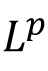spaces, completeness ofand denseness results in, signed and complex measures, Radon-Nikodym theorem, Lebesgue decomposition theorem, dual ofspaces, dual of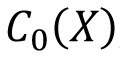, product measures, Fubini's theorem and its application, differentiation of measures. Reference materials: P. R. Halmos: Measure Theory. D. Van Nostrand Company, Inc., New York, 1950. Walter Rudin: Real and complex analysis, McGraw-Hill international editions. Credits: 9

 Syllabus: Pre-requisite: MTH301 / Instructor's consent Fourier transform on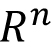,andtheory, Complex interpolation,theory, Paley-Wiener theorem, Wiener-Tauberian theorem, Hilbert transform, Maximal function, real interpolation,Riesz transform, transference principle, Multipliers and Fourier Stieljes transform, Calderon-Zygmund singular integrals, Littlewood-Paley theory. Reference materials: Credits: 9

 Syllabus: Pre-requisite: MTH 301 / Instructor's consent Differentiable manifolds; Tangent space. Vector fields; Frobenius theorem; Relation between Lie sub-algebras & Lie subgroups; Cartans theorem on closed subgroups; One parameter subgroups; Exponential maps; Adjoint representation; Homogeneous spaces; Compact Lie groups; Symmetric spaces Reference materials: Credits: 9

 Syllabus: Pre-requisite: MTH 301 / Instructor's consent Fourier Analysis: A review, Convolutions, Multipliers and Filters, Poisson Summation Formula, Shannon Sampling Discrete Fourier Transform, Fast Fourier Transform, Discrete Wavelets, Continuous Wavelets, Uncertainty Principles, Radar Ambiguity, Phase Retrieval, Random Transform, Basic Properties, Convolution and Inversion, Computerized Tomography Reference materials: Credits: 9

 Syllabus: Pre-requisite: MTH 304 / Instructor's consent Classification of 2dimensional surfaces; Fundamental group; Knots and covering spaces; Braids and links; Simplicial homology groups and applications; Degree and Lefschetz Number, Borsuk Ulam Theorem, Lefschetz Fixed Point Theorem Reference materials: Credits: 9

 Syllabus: Pre-requisite: MTH 301 / Instructor's consent Best approximation in normed spaces, Tchebycheff systems, Tchebycheff Weierstrass Jackson Bernstein Zygmund Nikolaev etc. theorems, Fourier series, Splines, Convolutions, Linear positive, Variation diminishing, Simultaneous etc. approximations. Direct inverse saturation theorems, Applications. Reference materials: Credits: 9

 Syllabus: Pre-requisite: MTH 301 / Instructor's consent Unbounded Operators, Matrix representation, Self-adjointness Criterion, Quadratic Forms, Differential Operators, Self-adjoint Extensions, Functional Calculus, Spectra of Self-adjoint Operators, Semi analytic vectors, Theorems of Nelson and Nussbaum, States and Observables, Super selection Rules, Position and Momentum, An Uncertainty Principle of Bargmann, Canonical Commutation Relations, Schrodinger representations, Schrodinger Operators, Self-adjointness, A Theorem of Kato, Spectral Theory for Schrodinger Operators, Discrete Spectrum, Essential Spectrum Reference materials: N. Akhiezer and I. Glazman: Theory of Linear Operators in Hilbert Space II, Dover, 1961. J. Blank, P. Exner and M. Havlivcek: Hilbert Space Operators in Quantum Physics, Springer, 2008. T. Kato: Perturbation Theory, Springer, 1976. M. Miklavcic: Applied Functional Analysis and Partial Differential Equations, World Scientific, 1998. M. Reed and B. Simon: Methods of Modern Mathematical Physics II, Academic Press, 197 Credits: 9

 Syllabus: Pre-requisite: MTH 301 / Instructor's consent Models of Hyperbolic Space: Upper Half Space Model & Disc Model; Isometries of Hyperbolic Space; Geodesics; Slimness of Triangles and Exponential Divergence of Geodesics in Hyperbolic Space; Isoperimetric Inequalities in Euclidean & Hyperbolic Space; Boundary of Hyperbolic Space; Review of Covering Spaces, Local Isometries and Fundamental groups; Properly Discontinuous Group actions; Fundamental Domains; Hyperbolic Surfaces Reference materials: R. Benedetti and C. Petronio: Lectures on Hyperbolic Geometry; Springer Verlag. B. Maskit: Kleinian Groups, Springer Verlag. M. R. Bridson and A Haefliger: Metric Spaces of Non-Positive Curvature, Springer. Svetlana Katok: Fuchsian Groups, The University of Chicago Press. B. H. Bowditch: A course on geometric group theory, preprint. Credits: 9

 Syllabus: Pre-requisite: MTH 301 / Instructor's consent Preliminaries, Elements of basis theory, Types of bases, Summability (summation of infinite series), Koethe sequence spaces, Bases in OTVS, Isomorphism theorems Reference materials: Credits: 9

 Syllabus: Pre-requisite(s): MTH 404, MTH 405 Introduction to Banach algebras: Gelfand Transform, commutative Banach algebras, Gelfand-Naimark Theorem, Spectral Theorem for normal operators and its applications to operators on a Hilbert space. The theory of Fredholm operators: spectral theory of compact operators (Fredholm Alternative). Operator matrices: Invariant and Reducing subspaces, the theory of ideals of compact operators (if time permits). Reference materials: J. B. Conway: A First Course in Functional Analysis Ron Douglas: Banach Algebra Techniques in Operator Theory Credits: 9

 Syllabus: Pre-requisite: MTH301 / Instructor's consent, Riesz Interpolation Theorem, Hilbert Transform, Hardy-Littlewood Maximal function,and BMO, Distributions, Important example of distributions, Calderon-Zygmund Distributions. Reference materials: E. M. Stein and R. Shakarchi: Functional Anlaysis, Princeton Lect. In AnalysisVol-4, 2011 W. Rudin: Functional Analysis, Tata-McGraw Hill Credits: 9

 Syllabus: Pre-requisite: MTH301 / Instructor's consent Banach Algebras and Spectral theory, Locally compact groups, Basic representation theory, Analysis on Locally compact abelian group, Analysis on compact groups, Group-algebra and structure of dual space. Reference materials: Credits: 9

 Syllabus: Pre-requisite: MTH 301 / Instructor's consent Topological linear spaces, Equicontinuity, Function spaces, Convexity & convex topological spaces, Hahn Banach theorem, Barrelled spaces, Principle of uniform boundedness, Bornological spaces, Duality theory (Mackey Arens Theorem, Mackey Topology, S-topology, Polarity) Reference materials: Credits: 9

 Syllabus: Pre-requisite: MTH301 / Instructor's consent Geometry of: Reinhardt Domains: Definition and Examples, Absolute spaces. Elementary Theory of SCV: Holomorphic Functions: Definition and Examples, C-R Equations and its Applications. Power Series: Definition and Examples, Abel's Lemma and its Consequences, Complete Reinhardt Domains: Definition and Examples, Cauchy's Integral Formula for Poly-discs and its Consequences. Some New Phenomena in SCV: Biholomorphic Inequivalence of Unit Polydisc and Unit Ball, Zeroes of holomorphic functions are never isolated, Logarithmically Convex and Complete Domains: Definition and Examples, Hartogs' Phenomenon: Continuation on Reinhardt Domains. Singularities of Holomorphic Functions: Analytic Sets: Definition and Examples, Riemann Removable Singularity Theorems and its Applications, Hartogs' Kugelsatz, Zero Set of a Holomorphic Function: Topological Properties. Local Properties of Holomorphic Functions: Weierstrass Preparation Theorem, Weierstrass Division Theorem, Ring of Germs of Holomorphic Functions is Noetherian and an UFD, Local Parametrization for the Zero Set of a Holomorphic Function. Domains of Holomorphy: Domains of Holomorphy: Definition and Examples, Various Notions of Convexity: Definition and Examples, Logarithmically Convex Complete Domains as Domain of Convergence, Theorem of Cartan and Thullen. Reference materials V. Scheidemann: Introduction to Complex Analysis in Several Variables, Birkhauser Verlag, Basel, 2005. R. Range: Holomorphic Functions and Integral Representations in Several Complex Variables, Springer-Verlag, New York, 1986. R. Narasimhan: Several Complex Variables, Univ. of Chicago Press, Chicago, 1995. L. Hormander: Introduction to Complex Analysis in Several Variables, North-Holland Publishing Co. Amsterdam, 1990. Credits: 9

 Syllabus: Pre-requisite: MTH201, MTH204 Definitions and first examples. Classical Lie algebras. Ideals and homomorphisms. Nilpotent Lie algebras. Engel's theorem. Solvable Lie algebras. Lie's theorem. Jordan Chevalley Decomposition. Radical and semisimplicity. The Killing form and Cartan's criterion. The structure of semisimple Lie algebras. Complete reducibility and Weyls theorem. Representation theory of the Lie algebra sl(2). Total subalgebras and root systems. Integrality properties. Simple Lie algebras and irreducible root systems. Reference materials: J. E. Humphreys: Introduction to Lie Algebras and Representation Theory, Berlin, New York, 1972. J. P. Serre: Complex semi-simple Lie algebras (translated from French: Algebras de Lie complex semi-simple). N. Jacobson: Lie algebras, Dover publication. Credits: 9

 Syllabus: Pre-requisite: MTH301, MTH 403 / Instructor's consent Subharmonic Functions: Subharmonic Functions: Definition and Examples, Maximum Principles, Montel's Theorem for Harmonic Functions, Harnack's Inequality, Montel's Theorem for Positive Harmonic Functions. Dirichlet Problem: Dirichlet Problem for Bounded Domains, Dirichlet Problem for Disc and Punctured Disc, Peron Method, Harmonicity of the Peron Solution, Sub harmonic Barrier, Riemann Mapping Theorem, Uniformization Theorem for Riemann Sphere. Green's Functions: Green's Formulae, Green's Function for Unit Disc and Complex Plane, Harmonic Measure, Green's unction for Domains with Analytic Boundary, Green's Function for General Domains. Riemann Surfaces: Abstract Riemann Surfaces: Definition and Examples, Finite Bordered Surfaces, Analytic and Meromorphic Functions on Surfaces: Definition and Examples, Harmonic Functions on Surfaces, Maximum Principles. The Uniformization Theorem: Green's Function of a Surface, Existence of Green's Function for Finite Bordered Surfaces, Symmetry of Green's Function, Bipolar Green's Function, The Uniformization Theorem, Covering Surfaces. Reference materials T. Gamelin: Complex Analysis, Springer-Verlag, New York, 2004. C. Berenstein and R. Gay: Complex Variables. An Introduction, Springer-Verlag, New York, 1991. Credits: 9

 Syllabus: Pre-requisite: MTH301 / Instructor's consent Differentiable manifold, Tangent Spaces, Submanifolds, Immersions, Embeddings, Vector Fields, Riemannian Metric, Examples: Euclidean Metric & Hyperbolic Metric, Isometries and Local Isometries, Isometries of upper half plane. Affine connection, Covariant Differentiation, Parallel Vector Field, Parallel Transport, Levi-Civita Connection, Christoffel Symbols. Geodesics, Geodesics Flow, Exponential Map, Gauss Lemma, Normal Neighborhoods, Minimizing Properties of Geodesics, Convex Neighborhoods, Geodesics inhyperbolic plane. Curvature, Bianchi Identity, Sectional curvature. Jacobi Field, Jacobi Equation, Conjugate Points. Geodesic complete manifold, Hopf-Rinow theorem, Cartan Hadamard Theorem. Reference materials: M. P. Do Carmo: Riemannian Geometry, Birkhauser William M. Boothby: An Introduction to Differentiable Manifolds and Riemannian Geometry, Academic Press Kumaresan: Differential geometry and Lie groups, TRIM Series Credits: 9

 Syllabus: Pre-requisite: MTH301 / Instructor's consent Recalling definition of homotopy and fundamental group. Van Kampen theorem.Free groups and Free product of groups. Fundamental groups of sphere, Wedgeof circles. CW-complexes definition and examples. Statement of classification of compact surfaces and polygonal representation. Computation of fundamental groups of compact surfaces. Homotopy extension property of a CW pair. Proof of the fact that if (X, A) has HEP and A contractible, then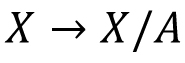is a homotopy equivalence. Examples from Hatcher. Covering spaces, Path lifting, homotopy lifting, general lifting. Examples of covering of wedge of circles, circles. Universal cover and existence of covering, classification of covering by subgroups of fundamental groups. Deck transformation,action of fundamental group on Universal cover, normal covering. Applications: Subgroup of free group is free, Cayley complexes. Homology: Singular homology; Theory (long exact, homotopy invariance and statement of excision, Mayer vietoris and computation for sphere, statement of Universal Coefficients Theorem) Applications: Degree of sphere and applications, homology and fundamental groups. CW homology, some computations of homology of projective spaces and surfaces, Euler characteristic and cells/ Betti numbers. Reference materials: Hatcher: Algebraic Topology Munkers: Algebraic Topology Massey: Basic course in Algebraic Topology Rotman: Algebraic Topology Armstrong: Basic Topology Bredon: Topology and Geometry Credits: 9

 Syllabus: Pre-requisite: None (Only for Ph.D. students of Statistics) Least upper bound principle; limits, monotone sequences; subsequences, Bolzano-Weierstrass, Cauchy sequences, completeness; countable and uncountable sets; convergence of series, conditional convergence; equivalence of completeness of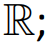; limsup, liminf, convergent series; absolute and conditional convergent, Riemann Rearrangement Theorem; convergence in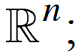; open sets and closed sets onCantor Intersection Theorem, Cantor set; limits and continuity; discontinuous functions; properties of continuous functions; uniform continuity; monotone functions; differentiation, Mean Value Theorem; Riemann integration; Fundamental Theorem of Calculus; sequence and series of functions, point wise convergence; uniform convergence, Weierstrass M-test, Dedekind test; uniform convergence and continuity; term by term integration and differentiation; power series; Taylor series, Weierstrass Approximation Theorem; analytic functions; Fourier series; differentiation of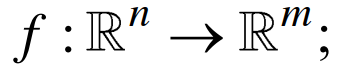partial derivatives; chain rule; higher derivatives, local extrema; Taylor expansion; multiple integrals, determinant and volumes, Jacobians.    Recommended books:  K.R. Davidson and A.P. Donsig: Real Analysis and Applications, Springer, 2010. R.S. Strichartz: The Way of Analysis, Jones and Bartlet Mathematics, 2000. Credits: 9

 Syllabus: Pre-requisite: MTH 421 / Instructor's Consent Volterra and Fredholm integral equations, Resolvent Kernels. Operator equations, Fredholm theory, Hilbert Schmidt theory. Nonlinear integral equations, Singular integral equation Reference materials: Credits: 9

 Syllabus: Pre-requisite: MTH 421 / Instructor's Consent Elements of operator theory and Hilbert spaces; Introduction to the theory of distributions. Sobolev Spaces: Imbedding and compactness theorems, Fractional spaces and elements of trace theory. Applications to elliptic equations or parabolic equations Reference materials: Credits: 9

 Syllabus: Pre-requisite: MTH201 / Instructor's Consent Basic results on system of linear equations, Cauchy-Binet formula for computing determinant, Rank factorization of a singular matrix, Vector Spaces associated with a matrix, Different types of generalized inverses, Moore-Penrose Inverse. Spectral Theorem of symmetric matrices, algebraic and geometric multiplicities, characteristic and minimal polynomials, Courant-Fischer Theorem, Interlacing theorems for eigenvalues, Quadratic forms, Positive definite matrices and its characterizations. Graphs, digraphs, examples, sub-graphs, simple graphs, emphasis on tree, cycle graph and complete graph/bipartite graphs, complement of a graphs, path, walk and cycle in a graph. Definition, example, rank and minor of {0, 1,-1}-incidence matrix, Substructure of a graph, Path-matrix and Moore-Penrose inverse of incidence matrix. Adjacency matrix, relationship with number of edge/walks/..., eigenvalues of certain graphs, Determinant in terms of cycles and edges, Bounds on the spectrum of adjacency matrix, Laplacian matrix of a graph, it's properties, Matrix Tree theorem, Bounds on Laplacian spectral radius, Perron Frobenius theory, Basic results on Regular and Strongly regular graphs, Algebraic connectivity, Fiedler's theorems, Bounds on algebraic connectivity. Reference materials: R. B. Bapat: Graphs and Matrices, TRIM Series, Hindustan Book Agency, New Delhi, 2010. C. Godsil and G. Royle: Algebraic Graph Theory, GTM, Springer, 2001. N. Biggs: Algebraic Graph Theory, Cambridge Mathematical Library, 2016. Credits: 9

 Syllabus: Pre-requisite: MTH 421 / Instructor's Consent Picard's theorem, Boundedness of solutions, Omega limit points of bounded trajectories. LaSalle's invariance principle; Stability via Lyapanov's indirect method, Converse Lyapanov functions, Sublevel sets of Lyapanov functions, Stability via Lyapanov's direct method, Converse Lyapanov's theorems, Brokett's theorem, Applications to control system; Stable and unstable manifolds of equilibria, Stable manifold theorem, Hartman Grobman theorem, Examples and applications, Center manifold theorem, Center manifold theorem, Normal form theory, Examples and applications to nonlinear systems and control; Poincare map, and stability theorems for periodic orbits; Elementary Bifurcation theory. Reference materials: Credits: 9

 Syllabus: Pre-requisite: MTH 405, MTH 424 / Instructor's Consent Review of topics in Functional Analysis and Sobolev Spaces, Mapping between Banach Spaces, Degree theory, Bifurcation theory, Variation method, Constrained critical points, deformation and Palais condition, Linking thorems, Mountain pass theorem and Ekeland's variation principle. Reference materials: H. Brezis: Functional Analysis- Sobolev and Partial Differential Equations, Universitext Springer L. Nirenberg: Topics in Nonlinerar Functional Analysis, Courant Institute of Mathematical Sciences. D. G. De Figueiredo: The Ekeland Variational Principle with Applications and Detours, TIFR Lecture Notes. A. Ambrosetti and A. Malchiodi: Nonlinear Analysis and Semilinear Elliptic Problems, Cambridge University Press. Credits: 9

 Syllabus: Pre-requisite: (MTH 201 or MTH204) or Instructors Consent. Theory of bilinear forms on vector spaces over arbitrary fields, isometry groups, Lie structure on the tangent spaces of isometry groups, revision of Lie algebras, semisimple Lie algebras, Chevalley basis, Cartan-Weyl classifica+on of semisimple Lie algebras, universal enveloping algebras, PBW, integral structures in Lie-algebras, construc+on of Chevalley groups, commuta+on rela+ons, classifica+on of Chevalley groups, automorphisms of Chevalley groups, introduc+on to elementary theory of schemes, chevalley groups as algebraic groups, Borel subgroups, Tori, Root datum and classifica+on of semisimple algebraic groups over algebraically closed fields. Reference materials: Hermann Weyl, Classical groups. R. Steinberg, Lectures on Chevalley groups, Lecture notes in Mathema+cs of AMS. A. Borel Linear algebraic groups GTM. Herman Weyl, Symmetry Princeton Science library. Credits: 9

 Syllabus: Pre-requisite(s): MTH 424/ Instructor's Consent Introduction to Semigroups, C0-semigroups, Hille-Yosida result, Heat equation, Wave equation, Inhomogeneous equations, Techniques for nonlinear problems, Palais-Smale Condition, Mountain Pass theorem, Hamilton Jacobi equations, Viscosity Solutions. Credits: 9

 Syllabus: Pre-requisite: MTH611/612/613 Background from commutative algebra: Local rings, localizations, primary decomposition, Integral extensions, integral closures. Algebraic geometry: Affine algebraic sets, Hilbert Nullstellansatz, Projective algebraic sets, projective Nullstellansatz, Affine varieties, structure sheaf, Prevarieties, varieties, morphisms, Affine and projective algebraic sets are varieties, dimension of varieties, products of varieties, images and fibers of morphisms, Tangent spaces, differential of a morphism, Smooth morphisms, smooth varieties, complete varieties. Algebraic Groups: Basic definitions and examples, Lie algebra of an algebraic group, Linear representations of algebraic groups, Affine algebraic groups are linear; connected projective algebraic groups are abelian varieties, rigidity of abelian varieties, quotients, Homogeneous spaces, Chevalley's theorem on algebraic groups (without proof). Reference materials: M. F. Atiyah and I. G. MacDonald: Introduction to commutative algebra. Michel Demazure and Peter Gabriel: Introduction to algebraic geometry and algebraic groups. T. A Springer: Linear Algebraic Groups. Armand Borel: Linear Algebraic Groups. David Mumford: Abelian varieties. R. Hartshorne: Algebraic Geometry. Credits: 9

 Syllabus: Pre-requisite: MTH 304, MTH 611 p-adic numbers: non-Archimedean absolute values, valuations, Ostrowski theorem, Cauchy sequences, p-adic integers, completions, Hensel's lemma, structure ofand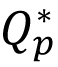. Quadratic forms: definition of quadratic forms and bilinear forms, equivalence of quadratic forms, local-global principle (Hasse-Minkowski theorem), rational points on conics. Infinite Galois Theory: Profinite groups and profinite topology, Infinite Galois extensions and Galois group as profinite groups, absolute Galois groups, the fundamental theorem of Galois theory (for infinite extensions), absolute Galois group of finite fields, Frobenius automorphism, absolute Galois group of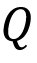and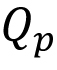. Geometry of curves over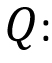Affine Varieties and projective varieties, curves and function fields, divisors on curves, the Riemann-Roch theorem (statement without proof), Elliptic curves over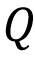, Group law on elliptic curves, Weierstrass equations, action of the absolute Galois group of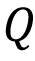overpoints of elliptic curves, Weak Mordell-Weil Theorem, Mordell-Weil Theorem, Faltings' Theorem (statement without proof). Reference materials: Neal Koblitz: p-adic numbers, p-adic analysis and zeta-functions, 2nd ed, GTM, vol 58, Springer-Verlag. Serge Lang: Algebra, 3rd ed, GTM, Vol. 211, Springer-Verlag. J.-P. Serre: A course in arithmetic, Springer-Verlag, 1973, Translated from French; GTM, No. 7. Joseph H. Silverman, The arithmetic of elliptic curves, GTM, vol. 106, Springer-Verlag, 1992. Corrected reprint of the 1986 original. William Fulton, Algebraic Curves: An Introduction to Algebraic Geometry, 2008, http://www.math.lsa.umich.edu/wfulton/CurveBook.pdf. Credits: 9

 Syllabus: Pre-requisite(s): MTH 301, MTH 404/ Instructor's Consent Harmonic analysis on: Fourier Transform, basic properties, inversion formula, Plancherel formula, Paley-Wiener theorem, Young's inequality. Tangent space of, inner product on the tangent spaces of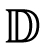,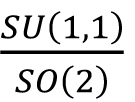as a Riemannian symmetric space, geodesics on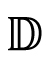, horocycles. Iwasawa decomposition, Cartan decompostion of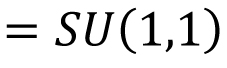, Haar measures in these decompositions, unimodular group. Laplace-Beltrami operator onand its eigenfunctions, the Helgason-Fourier transform, Radon transform, elementary spherical functions, spherical transform, Abel transform, assymptotics of elementary spherical functions. Inversion and Plancherel formula for Helgason-Fourier transform, Paley-Wiener theorem, Helgason-Johnson's theorem, Kunze-Stein phenomena. Reference material(s): R. Gangolli, and V. S. Varadarajan: Harmonic analysis of spherical functions on real reductive groups, Ergebnisse der Mathematik und ihrer Grenzgebiete [Results in Mathematics and Related Areas], 101. Springer-Verlag, Berlin, 1988. S. Helgason: Topics in harmonic analysis on homogeneous spaces, Progress in Mathematics, 13. Birkhäuser, Boston, Mass., 1981. S. Helgason: Groups and geometric analysis, Integral geometry, invariant differential operators, and spherical functions. Mathematical Surveys and Monographs, 83, AMS, Providence, RI, 2000. S. Helgason: Geometric analysis on symmetric spaces, Second edition. Mathematical Surveys and Monographs, 39, AMS, Providence, RI, 2008. R. A. Kunze and E. M. Stein: Uniformly bounded representations and harmonic analysis of the 2x2 real unimodular group, Amer. J. Math. 82, 1—62, 1960. Credits: 9

 Syllabus: Pre-requisite: MSO201 / Instructor's consent Brief review of simple and multiple linear regression along with the outlier detection methods. Basic idea of non-parametric regression. Measures of robustness in different statistical problems (e.g., the influence function and the breakdown point). Least squares and least absolute deviations in regression model; Least median squares and least trimmed squares estimators; Different statistical properties and the computational algorithms of the robust estimators of the location and the scale parameters (for the univariate as well as the multivariate data); Robust measure of association and robust testing of hypothesis problems. Data-depth and the robust estimators based on the data-depth; Multivariate quantiles and its properties along with the computational algorithm; Possible extension of the depth-based and the quantile-based estimators for the functional data. Some applications of robust estimators (e.g., robust classification and cluster analysis), Robust model selection problems. Reference materials: P J Rousseeuw and A M Leroy: Robust Regression and Outlier Detection, Wiley, 2003 P J Huber: Robust Statistics, Wiley 2009 A W van der Vaart: Asymptotic Statistics, 2000 R J Serfling: Approximation Theorems of Mathematical Statistics, Wiley, 2002 B. W. Silverman: Density Estimation for Statistics and Data Analysis, Chapman and Hall, 1999 Credits: 9

 Syllabus: Pre-requisite(s): MTH 405/ Instructor's Consent Existence of Minimizer, Perron's Method variational form, Concentration-Compactness, Ekeland's Variational Principle, Palais-Smale Condition and Mountain Pass theorem, Application to semilinear equation with symmetry, Krasnoselskii Genus, Ljusternik-Schinelman Category, Multiple critical points of even functional on symmetric manifolds, Pohozaev Non-Existence Result, Brezis-Nirenberg result, Nehari Manifold and Fibering Method, Alexandrov's Moving Plane and Berestycki Sliding Method. Reference material(s): Michel Willem: Minimax Theorems, Birkhauser A. Ambrosetti and G. Prodi: A primer of Nonlinear Analysis, Cambridge studies in advanced mathematics. A. Ambrosetti and A. Malchiodi: Nonlinear Analysis and semilinear elliptic problems, Cambridge studies in advanced mathematics. Credits:

 Syllabus: Pre-requisite(s): MTH 201, MTH 304, MTH 305/ Instructor's consent Definition of manifolds and the fundamental ideas connected with them: Local coordinates, topological manifolds, differentiable manifolds, tangent spaces, vector fields, integral curves of vector fields and one-parameter group of local transformations, define manifold with boundary and orientation of a manifold. Differential forms on differentiable manifolds: Differential forms on Euclidean n-spaces and on a general manifold, the exterior algebra, Interior product and Lie derivative, The Cartan formula and properties of Lie derivatives. Frobenius theorem. The de Rham Theorem: Homology of manifolds, Integral of differential forms and the Stokes theorem. de Rham cohomology, The de Rham theorem. Applications of the de Rham theorem; Hopf invariant, the Massey product, cohomology of compact Lie groups, Mapping degree, Integral expression of the linking number by Gauss. Differential forms on Riemannian manifolds: The *- operator of Hodge, Laplacian and harmonic forms. The Hodge theorem and the Hodge decomposition of differential forms. Applications of the Hodge theorem. Reference material(s): Shigeyuki Morita: Geometry of Differential Forms, Volume 201, Translations of Mathematical Monographs, AMS, Providence, RI, 2001. (Indian edition 2009). Frank W. Warner: Foundations of differentiable manifolds and Lie groups, volume 94 of Graduate Texts in Mathematics. Springer-Verlag, New York, 1983. Credits: 9

 Syllabus: Pre-requisite: MTH 416/ Instructor's Consent Brief review of topics in Multiple Linear Regression Analysis; Econometric tests on Heteroscedasticity and Autocorrelation; Restricted Regression; Errors in Variables; Functional Form and Structural Change; Stochastic Regressors; Instrumental Variable (IV) Estimation; Large Sample Properties of Least Square and IV estimators; Panel Data Models; Systems of Regression Equations -Seemingly Unrelated Regression Equations (SURE) & Multivariate Multiple Linear Regression; Simultaneous Equation Models - Structural and Reduced forms, Rank and Order conditions for Identifiability, Indirect Least Squares, 2-stage Least Squares and Limited Information Maximum Likelihood methods of estimation, k-class estimators and Full Information Maximum Likelihood Estimation; Models with lagged variables - Autoregressive Distributed Lag (ARDL) Models and Vector Autoregressive (VAR) Models; Topics on Econometric Time Series Models - Autoregressive and Generalized Autoregressive Conditionally Heteroscedastic (ARCH & GARCH) Models, Unit Root, Co-integration and Granger Causality. Credits: 9

 Syllabus: Pre-requisite: Instructor's Consent Examples from nature and laboratory, Role of spatio-temporal models in biology. Linear Stability analysis: Formulation, Normal modes, Application to system of one, two and more variables. Bifurcation analysis: Introduction, Saddle-node, Pitchfork, Transcritical, Hopf and Hysteresis bifurcations. Spatial pattern formation: Reaction-diffusion system, Turing instability, Pattern formation in reaction-diffusion system. Chemotaxis: Introduction, Modelling chemotaxis, Linear and Nonlinear analysis. Tumor modelling: Introduction, Models of tumor growth, Moving boundary problems, Response of immune system. Numerical simulation of Spatio-temporal model: Introduction, Finite-difference techniques, Monotone methods. Credits: 9

 Syllabus: Pre-requisite: MSO 201 / Instructor's Consent Decision function, Risk function, Optimal decision rules, Admissibility &completeness, The minimax theorem, The complete class theorem, Sufficient statistics. Invariant decision problems, Admissible & minimax invariant rules, The Pitman estimates, Estimation of a distribution function Credits: 9

 Syllabus: Pre-requisite: MSO 201 / Instructor's Consent Basic distribution theory, Moments of order statistics including recurrence relations, Bounds and approximations, Estimation of parameters, Life testing, Short cut procedures, Treatment of outliers, Asymptotic theory of extremes. Credits: 9

 Syllabus: Pre-requisite: MSO 201 / Instructor's Consent Introduction to simulation & Monte-Carlo studies; Generation of random variables. Interactive computational & graphical techniques in model building; Data based inference methods such as JackKnife, Bootstrap and cross validation techniques; Use of statistical packages in data analysis Credits: 9

 Syllabus: Pre-requisite: MSO 201 / Instructor's Consent Linear stationary processes, Auto covariance & spectral density functions &moving average processes, Linear non-stationary processes, Model estimation& identification, Forecasting, Transfer function models, Design for discrete control Credits: 9

 Syllabus: Pre-requisite: MSO 201 / Instructor's Consent Estimation methods, Commonly encountered problems in estimation, Statistical inference, Multi-response nonlinear model, Asymptotic theory, Computational methods. Reference materials: Credits: 9

 Syllabus: Pre-requisite: MTH 204, MTH 403 / Instructor's Consent Finite Fields, polynomial equations over finite fields; Chevalley-Warning theorem, Quadratic residue; law of quadratic reciprocity, p-adic numbers and p-adic integers, Quadratic forms (over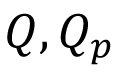and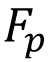). Riemann Zeta function and (Dirichlet) L-functions, Dirichlet's theorem on primes in arithmetic progression, Modular forms (for); relation with elliptic curves. Reference materials: J. P. Serre: A course in Arithmetic, GTM 07, Springer-Verlag. M. Ram Murty: Problems in Analytic Number Theory, GTM 206, Springer-Verlag. F. Diamond and J. Shurman: A first course in Modular forms, GTM 228, Springer-Verlag. H. Davenport: Multiplicative Number Theory, GTM 74, Springer-Verlag. J. Neukirch: Algebraic Number Theory, Grundlehren der Mathematischen Wissenschaften 322, Springer-Verlag. F. Gouvea: p-adic Numbers, an introduction, Universitext, Springer-Verlag. W. Scharlau: Quadratic and Hermitian forms, Grundlehren der Mathematischen Wissenschaften 270, Springer-Verlag. Credits: 9

 Syllabus: Pre-requisite: MSO 201/ Instructor's Consent Results of convergence in almost sure sense and in probability, DCT, Basic inequalities, Conditional expectation, Methods of resampling. Introduction to discriminant analysis, Bayes' risk, and its properties. Distance measures for density functions, and its relation with Bayes' risk. Empirical Bayes' risk and its convergence. Parametric methods: Maximum likelihood principle Fisher's linear discriminant function (LDA), quadratic discriminant analysis (QDA). Consistency results. Logistic regression, Linear support vector machines (SVM), Maximum linear separation and Projection pursuit. Non parametric methods: Kernel discriminant analysis (KDA), nearest neighbor classification (kNN), Universal consistency results. Idea of curse of dimensionality, and the use of dimension reduction techniques like random projections principal component analysis, etc. Semiparametric methods: Mixture Discriminant Analysis (MDA), Nonlinear SVM, Hybrid classifiers, Classification using data depth, Related consistency results. Reference materials: Richard O Duda, Peter E Hart and David G Stork: Pattern Classification, Wiley. Luc Devroye, Laszlo Gyorfi and Gabor Lugosi: A Probabilistic Theory of Pattern Recognition, Springer. Trevor Hastie, Robert Tibshirani, Jerome Friedman: The Elements of Statistical Learning: Data Mining, Inference, and Prediction, Springer. Credits: 9

 Syllabus: Pre-requisite: MTH309 or equivalent, MTH433 or equivalent and consent of instructor. Preliminaries: Different Modes of Convergence, Law of Large Numbers, Motivations. (6 Lectures) Function classes and their complexities, Glivenko-Cantelli class of functions. (8 Lectures) Symmetrization, Concentration Bounds. (4 Lectures) Vapnik-Cervonenkis (VC) classes of functions, Covering and Bracketing numbers, Examples: M-estimators. (8 Lectures) Donsker class, Uniform Central Limit Theorem, Examples. (6 Lectures) Arg-min continuous mapping theorem, Applications in Statistics: M-estimators, Lasso, Bootstrap consistency etc. (6 Lectures) More on Concentration Bounds/ Weak Convergence on Polish Spaces. (4 Lectures) Reference materials: A. W. van der Vaart and Jon A. Wellner. (1996). Weak Convergence and Empirical Processes, with Applications to Statistics. Springer Series in Statistics. Michael R. Kosorok. (2008). Introduction to Empirical Processes and Semiparametric Inference. Springer Series in Statistics. Sara van de Geer. (2009). Empirical Processes in M-Estimation. Cambridge Series in Statistical and Probabilistic Mathematics. Richard M. Dudley (2014). Uniform Central Limit Theorems. Cambridge Studies in Advanced Mathematics. G´abor Lugosi, Pascal Massart, and St´ephane Boucheron (2014). Concentration Inequalities: A Nonasymptotic Theory of Independence. Oxford University Press. Zhengyan Lin and Zhidong Bai. (2010). Probability Inequalities. Springer. Credits: 9

 Syllabus: Pre-requisite: None (Only for MS students) Credits: 9

 Syllabus: Pre-requisite: None (Only for MS students) Credits: 9

 Syllabus: Pre-requisite: None (Only for MS students) Credits: 9

 Syllabus: Pre-requisite: None (Only for MS students) Credits: 9

 Syllabus: Pre-requisite: MTH302 / Instructor's Consent Modal Propositional Logic - Models and Frames, Consequence relations, Normal modal systems. Invariance results for models, Bisimulations, Finite model property, Translation into First order logic. Frame definability and Second order logic, Definable and undefinable properties. Completeness, Applications. Algebraic Semantics - Lindenbaum-Tarski Algebras, Jonsson-Tarski Theorem, Duality, Goldblatt-Thomason Theorem. Modal Predicate Logic - Axiomatization, Barcan formula, Soundness and Completeness, Expanding domains; Identity. Examples of some other modal systems and applications - Temporal logic with Since and Until, Multi-modal and Epistemic Logics. Non-normal modal logics. Neighbourhood semantics. Reference materials: P. Blackburn, M. de Rijke and Y. Venema: Modal Logic, Cambridge Tracts in Theoretical Computer Science, 2001. G.E. Hughes and M.J. Cresswell: A New Introduction to Modal Logic, Routledge, 1996. B.F. Chellas: Modal Logic, Cambridge University Press, 1980. M. Fitting and R.L. Mendelsohn: First Order Modal Logic, Kluwer, 1998. Credits: 9

 Syllabus: Pre-requisite(s): Instructor's Consent Few Elements of Sobolev Spaces and Variational formulations, Besov spaces, Multi-scale Approximation and Multi-resolution, Elliptic Boundary Value Problems, Multi-resolution Galerkin Methods, Wavelets, Wavelet Galerkin Method, Adaptive Wavelet Methods, Wavelets on General Domains, Some Applications. Credits: 9

 Syllabus: Pre-requisite: MTH309A or equivalent, MTH431A or equivalent. Familiarity with Bayesian Analysis is preferred (MTH535A or equivalent), and consent of instructor. This course presents the theoretical and practical challenges of implementing a discrete-time general state space Markov chain Monte Carlo algorithm. Metropolis-Hastings, Gibbs samplers, and other component-wise algorithms are discussed in detail. The theoretical part of the course focuses on studying rates of convergence of Markov chains, and establishing the existence of a Markov chain central limit theorem. The practical challenges of implementing these algorithms, such as step-sizes, stopping criterion, output analysis, and implementation in statistical software are also discussed in detail. Reference materials: Meyn, Sean P., and Richard L. Tweedie. Markov chains and stochastic stability. Springer Science & Business Media, 2012. Nummelin, Esa. General irreducible Markov chains and non-negative operators. Vol. 83. Cambridge University Press, 2004. Brooks, Steve, Andrew Gelman, Galin Jones, and Xiao-Li Meng, eds. Handbook of Markov chain Monte Carlo. CRC press, 2011. Lindvall, Torgny. Lectures on the coupling method. Courier Corporation, 2002. Roberts, Gareth O., and Jeffrey S. Rosenthal. "General state space Markov chains and MCMC algorithms." Probability surveys 1 (2004): 20-71. Jones, Galin L. "On the Markov chain central limit theorem." Probability surveys 1, no. 299-320 (2004): 5-1. Glynn, Peter W., and Ward Whitt. "The asymptotic validity of sequential stopping rules for stochastic simulations." The Annals of Applied Probability 2, no. 1 (1992): 180-198. Roberts, Gareth O., and Jeffrey S. Rosenthal. "Optimal scaling for various MetropolisHastings algorithms." Statistical Science16, no. 4 (2001): 351-367. Jarner, Søren Fiig, and Ernst Hansen. "Geometric ergodicity of Metropolis algorithms." Stochastic Processes and their Applications 85, no. 2 (2000): 341-361.

 Syllabus: Pre-requisite: Instructor's Consent Approximation of functions, Numerical quadrature, Methods of numerical linear algebra, Numerical solutions of nonlinear systems and optimization, Numerical solution of ordinary and partial differential equations. Reference materials: E Isaacson and H Bishop: Analysis of Numerical Methods, Dover Publications, 1994 J Stoer and R Bulirsch: Introduction to Numerical Analysis, Springer-verlag, 1980. Credits: 9

 Syllabus: Pre-requisite: MTH611/ 612/ 613 / Instructor's Consent A brief review of commutative algebra - localization, Noetherian rings and modules, integral extensions, Dedekind domains and discrete valuation ring, Spec of a ring. Number field, ring of integer, primes and ramifications, class group, finiteness of class number, Dirichlet's unit theorem, global fields, local fields, valuations (Time permitting: Cyclotomic fields, zeta functions and L-functions, class number formula, adeles and ideles). Reference materails: D. Marcus: Number Fields, Universitext, Springer-Verlag. R. Narasimhan et. al.: Algebraic Number theory, TIFR Pamphlet, www.math.tifr.res.in/~publ/pamphlets/index.html E. Jody and M. Ram Murty: Problems in Algebraic Number Theory, GTM 190, Springer-Verlag. J.S. Milne: Algebraic Number Theory, Course notes, available at http://www.jmilne.org. J. Neukirch: Algebraic Number Theory, Grundlehren der Mathematischen Wissenschaften 322, Springer-Verlag. S. Lang: Algebraic Number Theory, GTM 110, Springer-Verlag. J P Serre: A course in Arithmetic, GTM 7, Springer-Verlag. M F Atiyah and I G Macdonald: Introduction to commutative algebra, Addison-Wesley Publ. Co. Credits: 9

 Syllabus: Pre-requisite: MTH 201, MTH 405 / Instructor's Consent Compact Operators, Fredholm Alternative, Fredholm Operators, The Fredholm Index and the Abstract Index, Equality of Fredholm Index and Abstract Index, Essential Spectrum, Toeplitz Operators, Fredholm Theory for Toeplitz Operators, Connectedness of the Essential Spectrum Reference materials J. Conway: A course in Functional Analysis, Springer, 2006. R. Douglas: Banach Algebra Techniques in Operator Theory, Springer, 1998. M. Miklavcic: Applied Functional Analysis and Partial Differential Equations, World Scientific, 1998. Credits: 9

 Syllabus: Pre-requisite: Instructor’s Consent Overview of Riemannian Geometry: Definition of Manifolds, Diffeomorphism, Sard’s Theorem, Partition of Unity, Whitney embedding theorem, Tangent spaces and Tensor fields, Integration on Manifold, Stokes Theorem, Metric Tensor, Hodge Star Operation, Stokes theorem for Riemannian Manifold, Lie Derivative, Connection, Geodesic, Riemannian Curvature, Ricci tensor, Bianchi Identity.  Sobolev Spaces in Riemannian Manifold: Definitions, Density, Imbedding Theorem, Trace Theory.  Linear PDE in Riemannian Manifold: Existence theory of Poisson equation, Harmonic Forms, Hodge Decomposition, Spectral theory, Gromov’s Isoperimetric Inequality, Green’s Function of the Laplacian.  Reference materials: Some Nonlinear Problems in Riemannian Geometry, Thierry Aubin, SMM, Springer, 1982. Sobolov Spaces in Riemannian Manifold, Emmanuel Hebey, LNM, Vol-1653, Springer Credits: 9

 Syllabus: Pre-requisite: MTH 424/ Instructor's Consent Periodic composite materials (one dimensional and layered materials), Homogenization of second order el- liptic equations: periodic case, Tartar's method of oscillating test functions; Convergence of energy and correctors, Method of Two-scale convergence and convergence result, Homogenization in the non-periodic case (G-convergence and H-convergence), Optimal bounds: Hashin-Shtrikman. Credits: 9

 Syllabus: Pre-requisite: Instructor's Consent Introduction to integral equations, degenerate kernel method, projection methods, They Nystrom method, Solving multivariable integral equations, integral equations on a smooth and piecewise smooth planar boundary, boundary integral equation in 3 dimensions. Reference materials: Kendall E. Atkinson: The numerical solution of integral equations of the second kind, Cambridge University Press. Rainer Kress: Linear Integral Equations, Springer. Credits: 9

 Syllabus: Pre-requisite: MTH 424/ Instructor's Consent Introduction to Finite Difference Method (FDM), General Theory of FDM, FDM for 1-DEquations, Explicit FDM for One‐Factor Models, Trinomial method for Barrier Options, Exponentially Fitted FDM for Barrier Options, Computational issues in Barrier and Look-back option models, FDM for One‐Factor Black‐Scholes Equation, Implicit‐Explicit Method for Jump Process in Black Scholes Equations, FDM for Multi‐Factor Instrument Pricing, Heston Model, Asian Option, Multi‐Asset option, Front fixing Method, American Option. Credits: 9

 Syllabus: Pre-requisite: MTH 649 Algebraic Topology-I (Fundamental groups, covering spaces, Homology theory), Knowledge of cohomology is desirable/ Instructor consent. Week 1 CW complexes, higher homotopy groups Week 2 Relative homotopy groups,properties, action of fundamental group. Week 3 Long exact sequence of a pair, Whitehead Theorem. Week 4 Cellular and CW approximation. Week 5 Postnikov Towers, k-invariants, Whitehead towers. Week 6 Freudenthal Suspension Theorem, Homotopy Excision Theorem and computations. Week 7 Moore spaces and Eilenberg–MacLane space. Week 8 Hurewicz Theorem. Week 9 Homotopy Lifting Property, Fibrations, fiber bundles. Week 10 Long Exact Sequences for fibrations, applications to spheres. Week 11 Whitehead products, stable homotopy groups, ring structures. Week 12 Loop spaces & Suspension, exact and co-exact Puppe sequences Week 13 Relations to cohomology theory. Week 14 Obstruction Theory. Reference materials: A. Hatcher: “Allen Hatcher”, Cambridge Univ Press. G. W. Whitehead: “Elements of Homotopy Theory”, GTM 61, Springer 1978. G. Bredon: “Geometry and Topology”, GTM 139, Springer 1993. A. Dold: “Lectures in Algebraic Topology”, Classics in Mathematics, Springer 1995. P. May: “A Concise Course in Algebraic Topology”, University of Chicago Press, 1999. Credits: 9

 Syllabus: Pre-requisite: MTH 424/ Instructor's Consent Introduction to Image Processing; Mathematical Preliminaries–Direct Method in Calculus of Variation, Space of functions of Bounded Variations, Viscosity solutions of PDEs, Elements of Differential Geometry; Image Restoration‐The Energy Method, PDE‐Based Methods Smoothing & Enhancing PDEs Linear & Nonlinear Diffusion models, Classical & Curvature based morphological process; The Segmentation Problem, The Mumford and Shah Functional, Geodesic Active Contours, Level set Method; Applications from computer vision– Sequence Analysis, Image Classification etc. Credits: 9

 Syllabus: Pre-requisite: MSO 201/ Instructor's Consent Measure Preserving Transformations, examples, flows, conditional probability and basic of topological groups. Ergodic Theorems, Ergodicity and Mixing. Almost everywhere convergence. Entropy, Selected topic from the following: Recurrence and Szemeredis Theorm/ Topological Dynamics/ Ornstein Theory/ Finitary coding between Bernoulli shifts and entropy invariance. Reference materials: P. Walters: Introduction to Ergodic Theory. K. E. Petersen: Ergodic Theory Ya. Sinai: Introduction to Ergodic Theory Credits: 9

 Syllabus: Pre-requisite: MSO 201/ Instructor's Consent Stochastic processes, Martingales and basic inequalities, Martingale convergence Theorem, Burkholders inequality and unconditional basis for. Banach space valued random variables, Gaussian and Rademacher, Levy's inequality, stable Random variables. Khintchine and Khintchine-Kahane inequalities, Hilbertian subspace of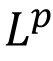, thin sets. Strong Law of Large Numbers, Law of iterated logarithm, Central Limit Theorem. Hilbertian section in unit ball of Banach space, John ellipsoid, K-convexity. Selected topic from: Type and co-type theory/ Random Fourier series/ Concentration of Measure phenomenon. Reference materials: M. Talagrand: Probability in Banach spaces. G. Pisier: Martingales in Banach spaces. G. Pisier: Volume of Convex Bodies and Geometry of Banach spaces Credits: 9

 Syllabus: Pre-requisite: MTH204/ Instructor's Consent Basic definitions and examples, Irreducible representations and Maschke's theorem, Constructing new representations, Schur's lemma, Characters and orthogonality relations, Regular Representation and decomposition, Number of irreducible representations of a group. Induced representation. Character table of some known groups. Representation theory of symmetric and alternating group. Algebraic integers and Burnside's pq theorem. Frobenius reciprocity formula, Group algebra and its decomposition, Mackey's irreducibility criterion. Artin's theorem on Induced characters, Elementary subgroups and Brauer's theorem. Reference materials: J.P. Serre: Linear representations of finite groups. Fulton; Harris: Representation theory: A first course. S. Lang, Algebra, GTM 211, Springer-Verlag. G. James and M. Liebeck, Representations and Characters of Groups, Cambridge University Press. Credits: 9

 Syllabus: Pre-requisite: MTH611/ 612/ 613 Linear groups: Topological groups; Linear groups (e.g.,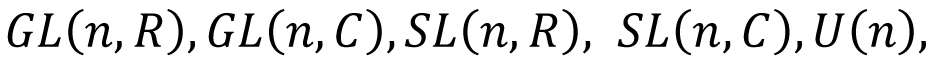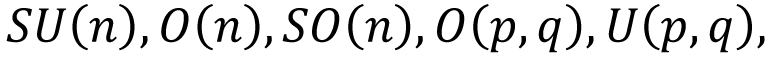, and their topological properties (e.g., compactness, connectedness) Exponential: Exponential of a matrix, Logarithm of a matrix. Linear Lie groups: One parameter subgroups; Lie algebra of a linear Lie group; Linear Lie groups are submanifolds; Campbell-Hausdorff formula. Lie algebra: Definitions and examples; Semisimple Lie algebras; Nilpotent and solvable Lie algebras. Representations of compact groups: Unitary representations; Schur orthogonality relations; Peter-Weyl theorem; character and central functions; absolute convergence of Fourier series; Casimir operator. Haar measure: Definition; differential forms and Haar measure on linear Liegroup; Unimodular group and examples. Analysis on SU(2): Haar measure on SU(2); irreducible representations of SU(2);Laplace operator on SU(2); Fourier series on SU(2); Heat equation on SU(2). Analysis on U(n): Highest weight theorem; Weyl integration formula; Character formula; Dimension formula; Laplace operator, Fourier series and Heat equation on U(n). Reference materials: S C Bagchi, S Madan, A Sitaram and U B Tewari: A first course on Representation theory and Linear Lie groups, University Press. Jacques Faraut: Analysis on Lie groups, Cambridge studies in advanced mathematics. B. C. Hall: Lie groups, Lie algebras and Representations, Springer GTM. J. Hilgert, K.-H. Neeb: Structure and Geometry of Lie groups, Springer Monograph in Mathematics. Credits: 9

 Syllabus: Pre-requisite: MTH 405/ Instructor's Consent Basics of Banach Algebras and C*-algebras, examples, spectrum. Commutative Banach Algebra and C*-algebras; maximal ideal spaces, Gelfand Transform; normal elements, continuous functional calculus. Representations of C*-algebras, von Neumann Algebras, WOT and SOT, density theorems, double commutant theorem. Spectral Theorem for normal operators. Abelian von Neumann algebras (Time permits: Type-I von Neumann algebras, factors, constructions of Type-II factors). Reference materials: Conway: Operator Theory. Davidson: C*-algebras by examples Takesaki: Operator Algebra, vol 1 Credits: 9

 Syllabus: Pre-requisite: Instructor's Consent Introduction, Test function spaces, Calculus with distributions, supports of distributions, Structure theorems, convolutions, Fourier transforms, L1(R), L2(R) theory of Fourier Transform, Tempered distributions, Paley Wiener theorem, Wiener Tauberian theorem, Applications of distributions theory and Fourier transform to differential equations Credits: 9

 Syllabus: Pre-requisite: MTH611/ MTH 612/ MTH 613 Definition of Lie group, Lie algebra and examples. Tangent Lie algebras Lie algebras associated to Lie groups. Correspondence between Lie algebras and simply connected Lie groups. The universal enveloping algebra of a Lie algebra and its properties. Hopf algebras and some basic Examples. Poincare-Birkhof -Witt theorem and deformations. Formal deformation of associative algebras. Formal deformation of Lie algebras. Cohomology of Lie algebras and its relation to deformation. Solvable, Nilpotent and semi-simple Lie algebras. Engel's theorem and Lie's theorem. The radical of a Lie algebra. Cartan criterion, Whitehead and Weyl theorems. Reference materials: William Fulton and Joe Harris: Representation theory, GTM-129, Springer- Verlag, New York, 1991. Dimitry Fuchs: Cohomology of infinite dimensional Lie algebras. Translated from the Russian by A. B. Sosinski. Consultants Bureau, New York, 1986. Susan Montgomery: Hopf algebras and their actions on rings, volume 82 of CBMS Regional Conference Series in Mathematics. Frank W. Warner: Foundations of differentiable manifolds and Lie groups, GTM-94, Springer- Verlag, New York, 1983. Credits: 9

 Syllabus: Pre-requisite: None (Only for Ph.D. students of Mathematics) Groups, Basic properties, Isomorphism theorems, Permutation groups, Sylow Theorems, Structure theorem for finite abelian groups, Rings, Integral domains, Fields, division rings, Ideals, Maximal ideals, Euclidean rings, Polynomial ring over a ring, Maximal & Prime ideals over a commutative ring with unity, Prime avoidance theorem and Chinese Remainder theorem, Field Extension, Cramer's rule, Algebraic elements and extensions, Finite fields. Determinants and their properties, Systems of linear equations, Eigenvalues and Eigenvectors, Caley Hamilton theorem, Characteristic and minimal polynomial, diagonalization, Vector spaces, Linear transformations, Inner product spaces Credits: 9

 Syllabus: Pre-requisite: None (Only for Ph.D. students of Mathematics) Calculus of Variations; Sturm Liouville Problem and Green's Function; Perturbation Methods and Similarity Analysis; Stability Theory. Credits: 9

 Syllabus: Pre-requisite: None (Only for Ph.D. students of Mathematics) Metric spaces, Open and closed sets, Compactness and connectedness, Completeness, Continuous functions (several variables and on metric spaces), uniform continuity C(X), X, compact metric space, Uniform convergence, compactness criterion, Differentiation, Inverse and Implicit function theorems. Riemann Integration, Lebesgue Integration, LP spaces. Complex Analysis: Analytic functions, Harmonic conjugates, Cauchy theorems and consequences, Power series, Zeros of analytic functions, Maximum modulus theorem, Singularities, Laurent series, Residues. Mobius transformations. Hilbert spaces: Inner product, Orthogonality, Orthonormal bases, Riesz Lemma, The space L2 as a Hilbert space Credits: 9

 Syllabus: Pre-requisite: None (Only for Ph.D. students of Statistics) Algebras and sigma algebras; Measurable spaces; Methods of introducing probability measures on measurable space; Random variables; Lebesgue integral; Expectation; Conditional probabilities and conditional expectations with respect to sigma algebras; Radon Nikodym theorem; Inequalities of random variables; Fubini's theorem; Various kinds of convergence of sequence of random variables; Convergence of probability measures; Central limit theorem; delta method; Infinitely divisible and stable distributions; Zero or One laws; Convergence of series; Strong law of large numbers; Law of iterated logarithm; Matringales and their basic properties Credits: 9

 Syllabus: Pre-requisite: None (Only for Ph.D. students of Statistics) Population and samples; Parametric and nonparametric models; Exponential and location scale families; Sufficiency and minimal sufficiency; Complete statistics; Unbiased and UMVU estimation; Asymptotically unbiased estimators; Method of moments; Bayes estimators; Invariance; Minimaxity and admissibility; The method of maximum likelihood; Asymptotically efficient estimation; Variance estimation; The jack knife; The bootstrap; The NP lemma; MLR; UMP tests for one and two sided hypotheses; Unbiased and similarity; UMPU tests in exponential families; Invariance and UMPI tests; LR tests; Asymptotic tests based on likelihoods; Chi-square tests; Bayes tests; Pivotal quantities; Inverting acceptance regions of tests; The Bayesian confidence interval; Prediction sets; Length of confidence intervals; UMA and UMAU confidence sets; Invariant confidence set. Credits: 9

 Syllabus: Pre-requisite: MTH 304, MTH 649, MTH 611/ 612/ 613 Definition of singular cohomology, axiomatic properties. Cup and cap product. Cross product and statements of Kunneth theorem and Universal coefficients theorem. Cohomology rings of projective spaces. Orientation of manifolds and Poincare duality. Definition of higher homotopy groups, homotopy exact sequence of a pair. Definition of bration, examples of brations, homotopy exact sequence of a bration, its application to computation of homotopy groups. Hurewicz homomorphism, The Hurewicz theorem. The Whitehead Theorem. Adjointness of loop and suspension, Eilenberg -Mac Lane spaces and cohomology (from Bredon). Reference materials: Hatcher: Algebraic Topology. Bredon: Topology and Geometry. Peter May: Concise course in Algebraic Topology. Tom Dieck: Algebraic Topology. Massey: Algebraic Topology: An Introduction Rotman: An Introduction to Algebraic Topology Credits: 9

 Syllabus: Pre-requisite: Instructor's Consent Smooth Manifolds, Vector bundles, Constructing New vector bundles Out of Old. Grassmann Manifolds and Universal bundles, The classification of vector bundles. Characteristic classes for vector bundles, Stiefel Whitney classes of manifolds, Characteristic numbers of manifolds, Thom spaces and the Thom isomorphism theorem, The construction of Stiefel Whitney classes, Chern, Pontryagin, and Euler classes. Reference materials: J. P. May: A concise course in Algebraic Topology. Chicago Lectures in Mathematics. University of Chicago Press, Chicago, IL, 1999. John W. Milnor and James D. Stasheff: Characteristic classes. Annals of Mathematics Studies, No. 76. Princeton University Press, Princeton, N. J.; University of Tokyo Press, Tokyo, 1974. Credits: 9

 Syllabus: Pre-requisite: Instructor's Consent Newton's Method for Nonlinear Equations, Numerical Integration of ODEs, Numerical Calculation of Eigenvalues, Numerical Solution of ODE Boundary-Value problems. Discrete Time Model: Numerical Detection of Fixed Points, Periodic Orbits, Bifurcation diagrams. Continuous Time Model: Continuation of Equilibrium Curves, Numerical Detection of Bifurcation Points of Co-Dimension One and Two, Local and Global Bifurcation Diagrams. Spatio-Temporal Model: Numerical Solution of Reaction-Diffusion Equations, Travelling Waves, Turing Patterns, Coupled Map Lattice Model. Delay Differential Equations: Numerical Solution of Delayed Temporal Model and Spatio-Temporal Model. Credits: 9

 Syllabus: Pre-requisite: MSO 201/ Instructor's Consent Introduction to pattern recognition supervised and unsupervised classification. Dimension reduction techniques: principal component analysis, multidimensional scaling features for maximum linear separation projection pursuit. Parametric methods for discriminant analysis: Fisher's linear discriminant function. Linear and quadratic discriminant analysis regularized discriminant analysis. Linear and nonlinear support vector machines. Cluster analysis: hierarchical and non-hierarchical techniques classification using Gaussian mixtures. Data depth: different notions of depth, concept of multivariate median, application of depth in supervised and unsupervised classification Credits: 9

 Syllabus: Pre-requisite: MSO 201/ Instructor's Consent Reliability concepts and measures, Components and systems, Coherent systems, Cuts and Paths, Modular decomposition, Bounds on system reliability; Life distributions, Survival functions, Hazard rate, Residual life time, Mean residual life function, Common life distributions, Proportional Hazard models; Notions of aging, Aging properties of common life distributions, closure under formation of coherent structures, Convolutions and mixture of these cases; Univariate and bivariate shock models, Notions of bivariate and multivariate and dependence; Maintenance and replacement policies, Availability of repairable systems, Optimization of system reliability with redundancy. Credits: 9

 Syllabus: Pre-requisite: MSO 201/ Instructor's Consent Multiple linear model, estimation of parameters under spherical and non-spherical disturbances by least squares and maximum likelihood methods, tests of hypothesis, R2 and adjusted R2. Prediction, within and outside sample predictions. Problem of structural change, tests for structural change. Use of dummy variable. Specification error analysis related to explanatory variables, inclusion and deletion of explanatory variables. Idea of Stein rule estimation. Exact and stochastic linear restrictions, restricted and mixed regression analysis. Multi collinearity, problem, implications and tools for handling the problem, ridge regression. Heteroskedasticity, problem and test, estimation under Heteroskedasticity. Autocorrelation, Durbin Watson test. Errors in variables, inconsistency of least squares method, methods of consistent estimation, instrumental variable estimation. Seemingly unrelated regression equation model, least squares, generalized least squares and feasible generalized least squares estimators. Simultaneous equations model, structural and reduced forms, rank and order conditions for identifiability, indirect least squares, two stage least squares and limited information maximum likelihood methods of estimation. Additional topics like as Panel data models and unit roots & co integration. Reference materials: Credits: 9

 Syllabus: Credits: 9